# RD Sharma Solutions For Class 7 Maths Chapter - 5 Operations On Rational Numbers

RD Sharma Solutions for Class 7 Maths Chapter 5 Operations On Rational Numbers are provided here. The students are provided with exercise-wise solutions to help them obtain a good score in the exam. The solutions are designed and well structured by the faculty at BYJU’S based on the latest syllabus of CBSE board. The problems are solved in an interactive manner to make the subject more interesting for the students. The students can use PDF of RD Sharma solutions while solving exercise wise problems, which boosts confidence while appearing for the exam. In this chapter, students will learn about addition, subtraction, multiplication and division of rational numbers.

## Download the pdf of RD Sharma Solutions For Class 7 Maths Chapter 5 Operations On Rational Numbers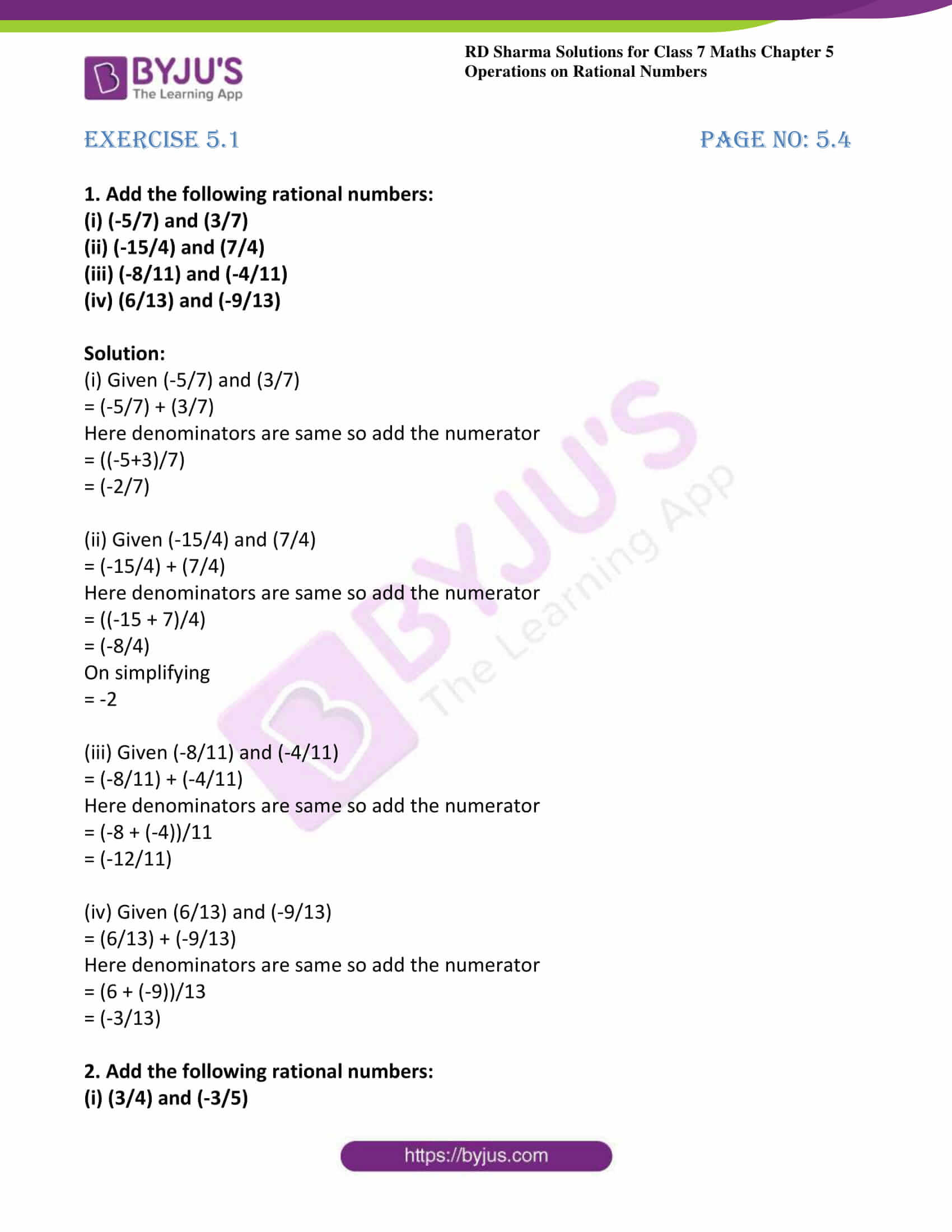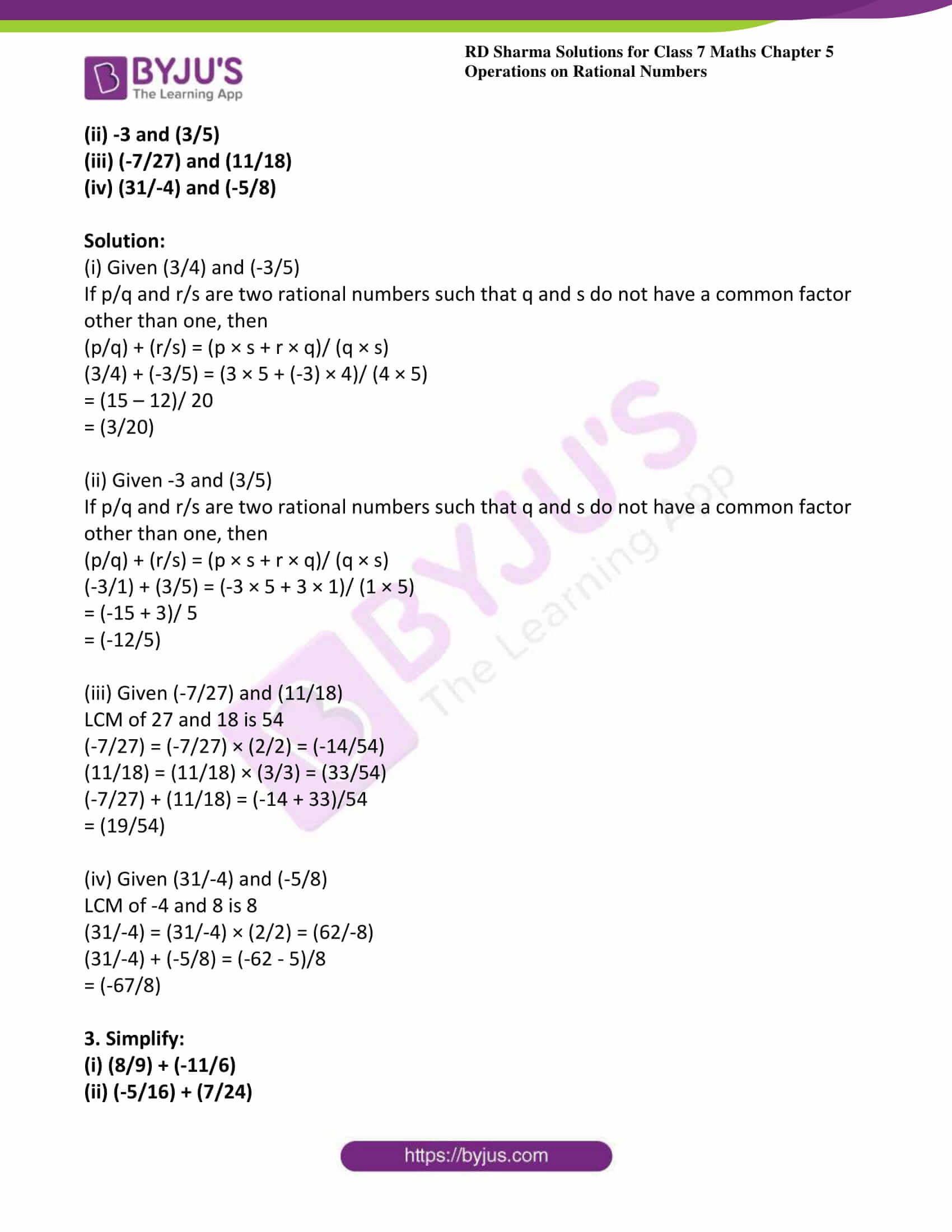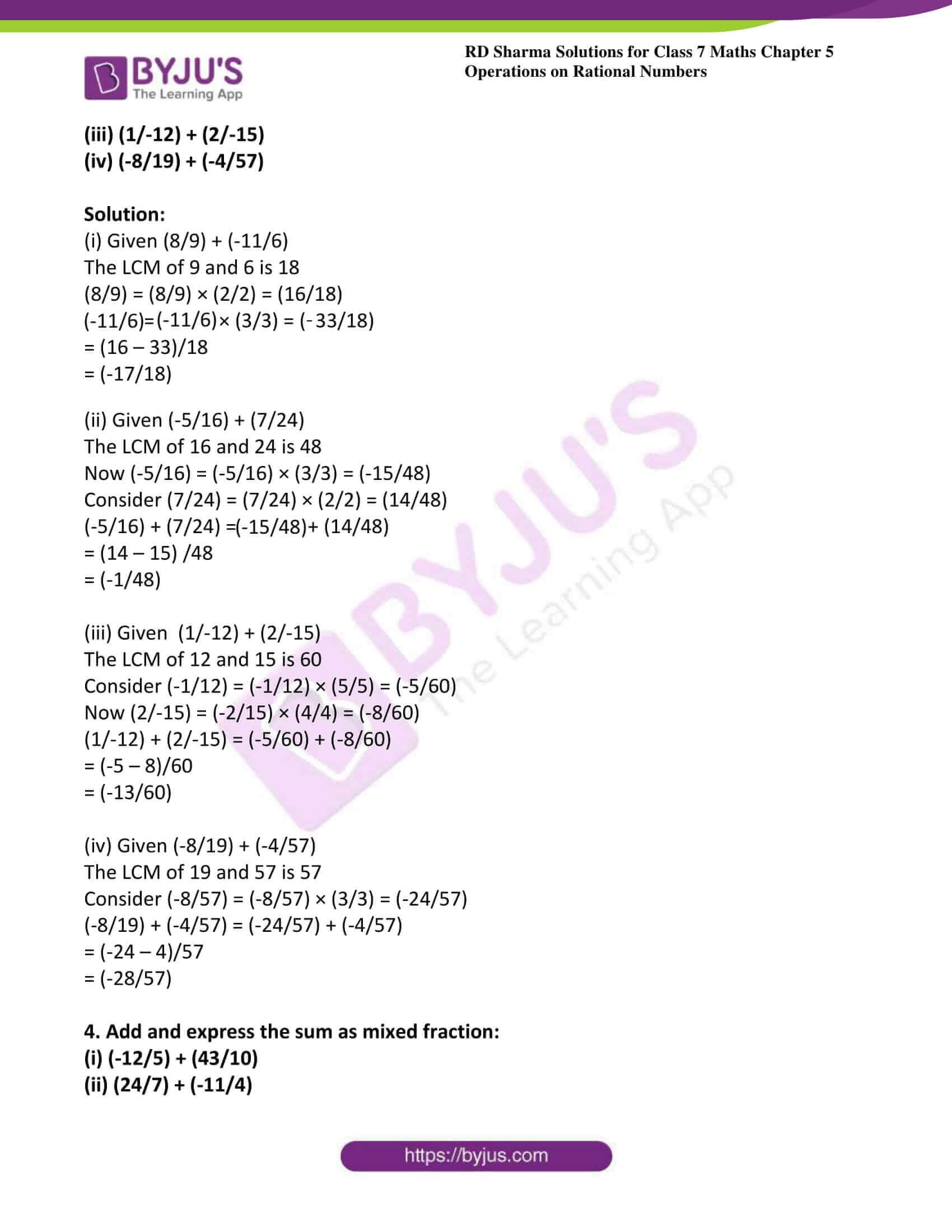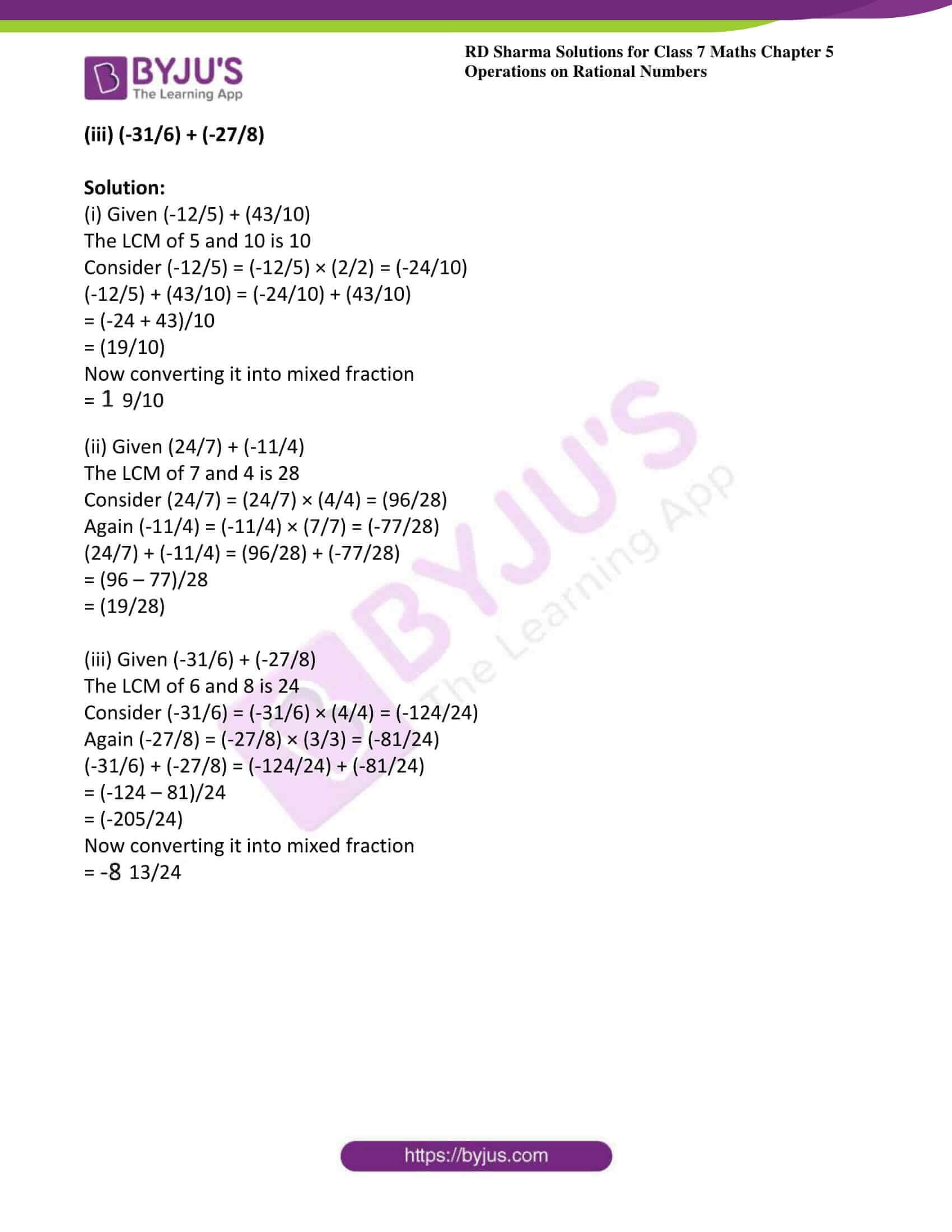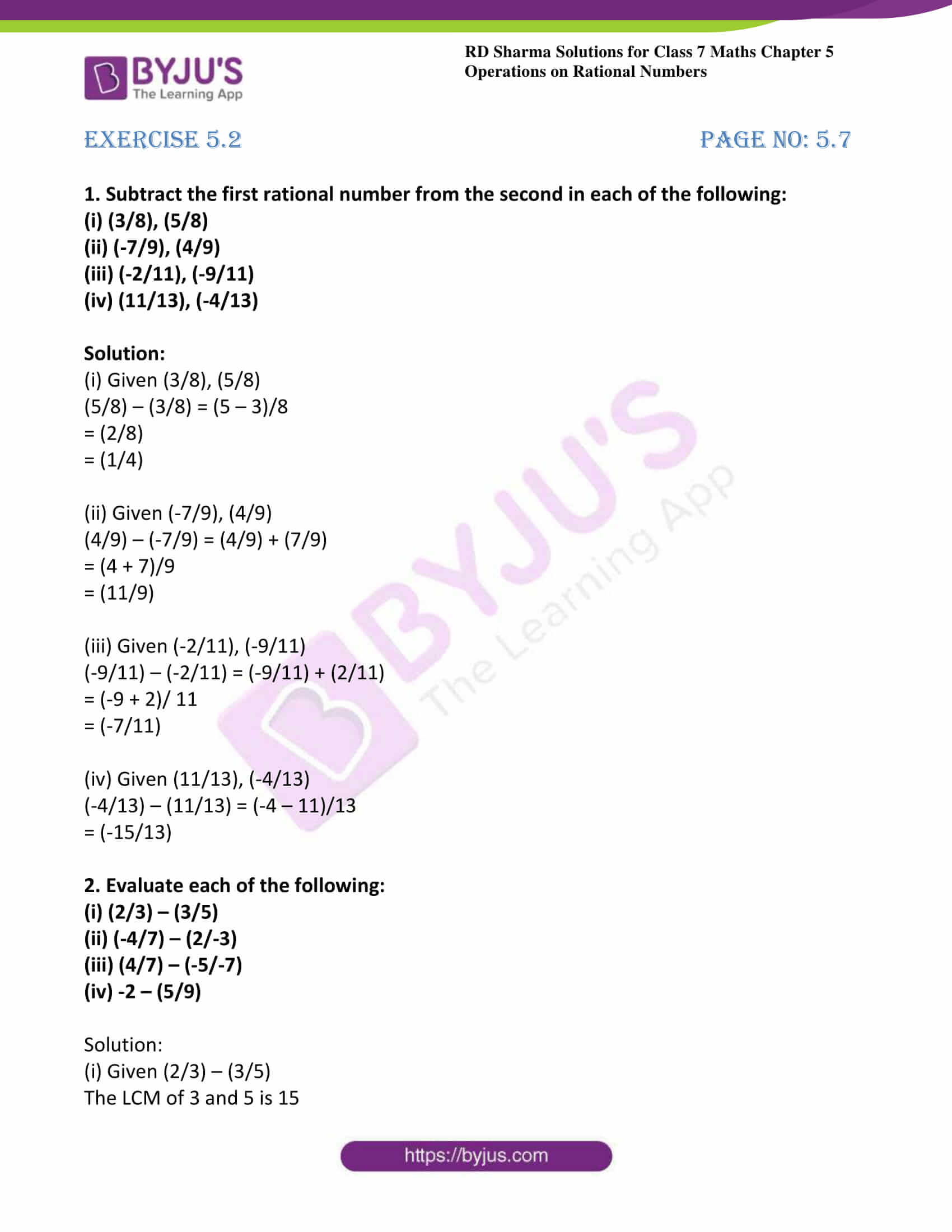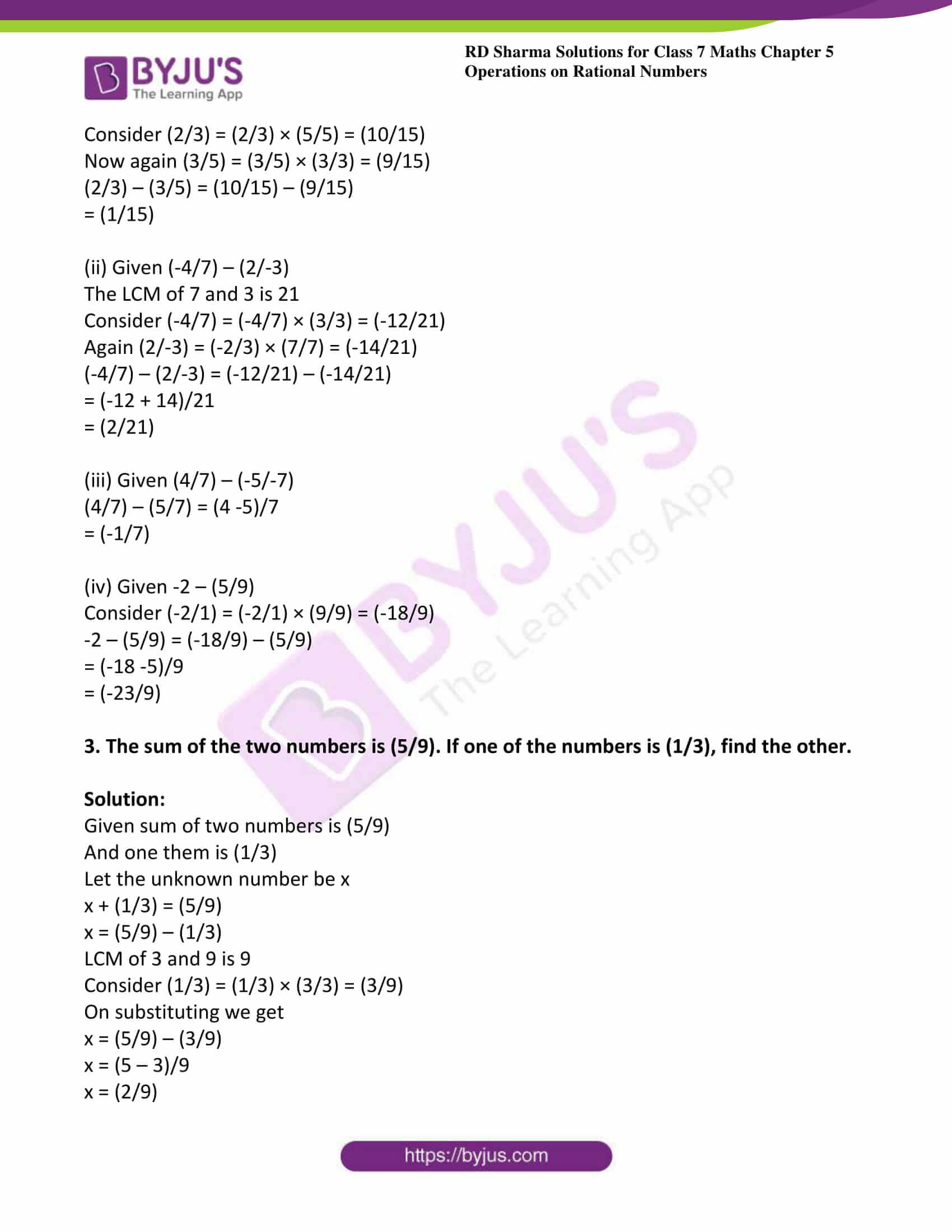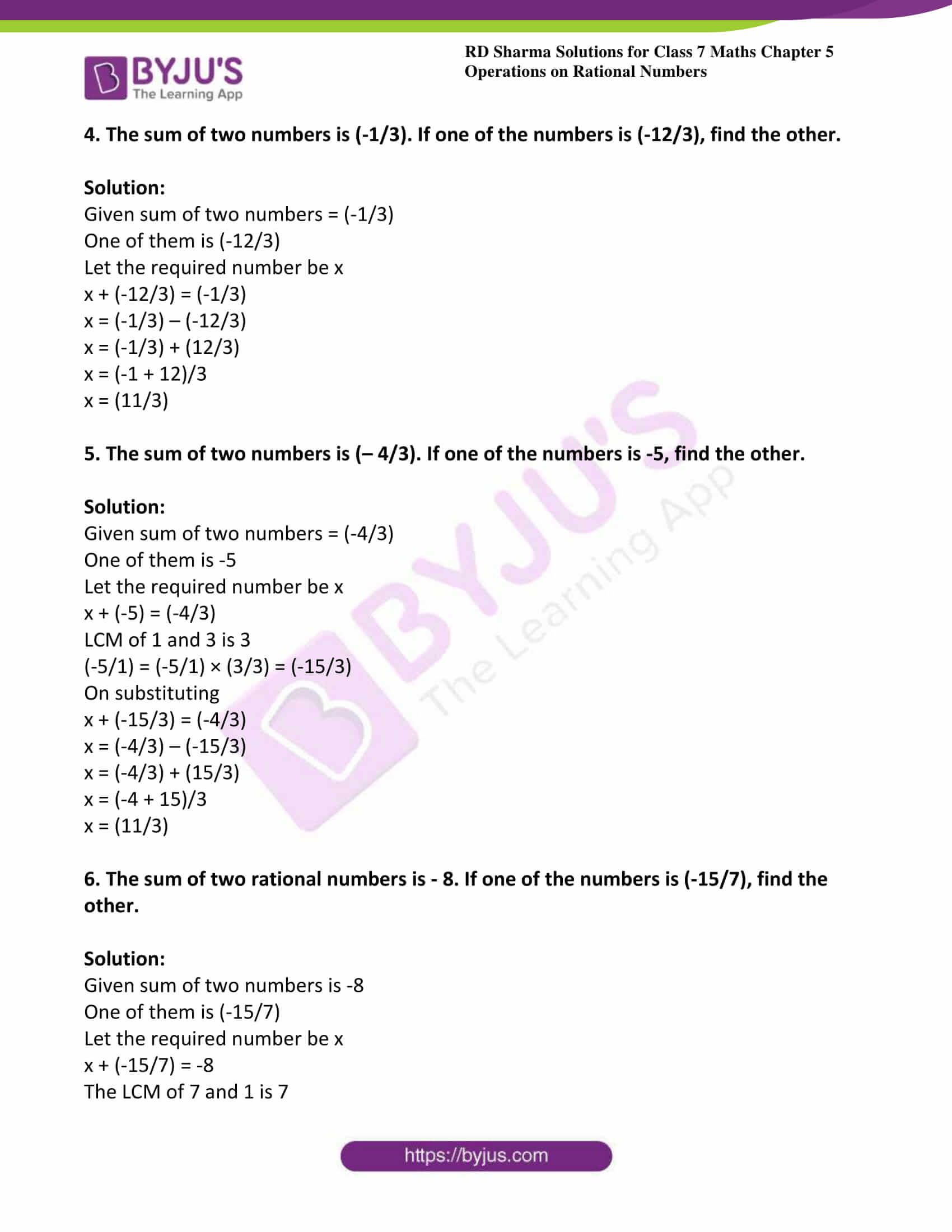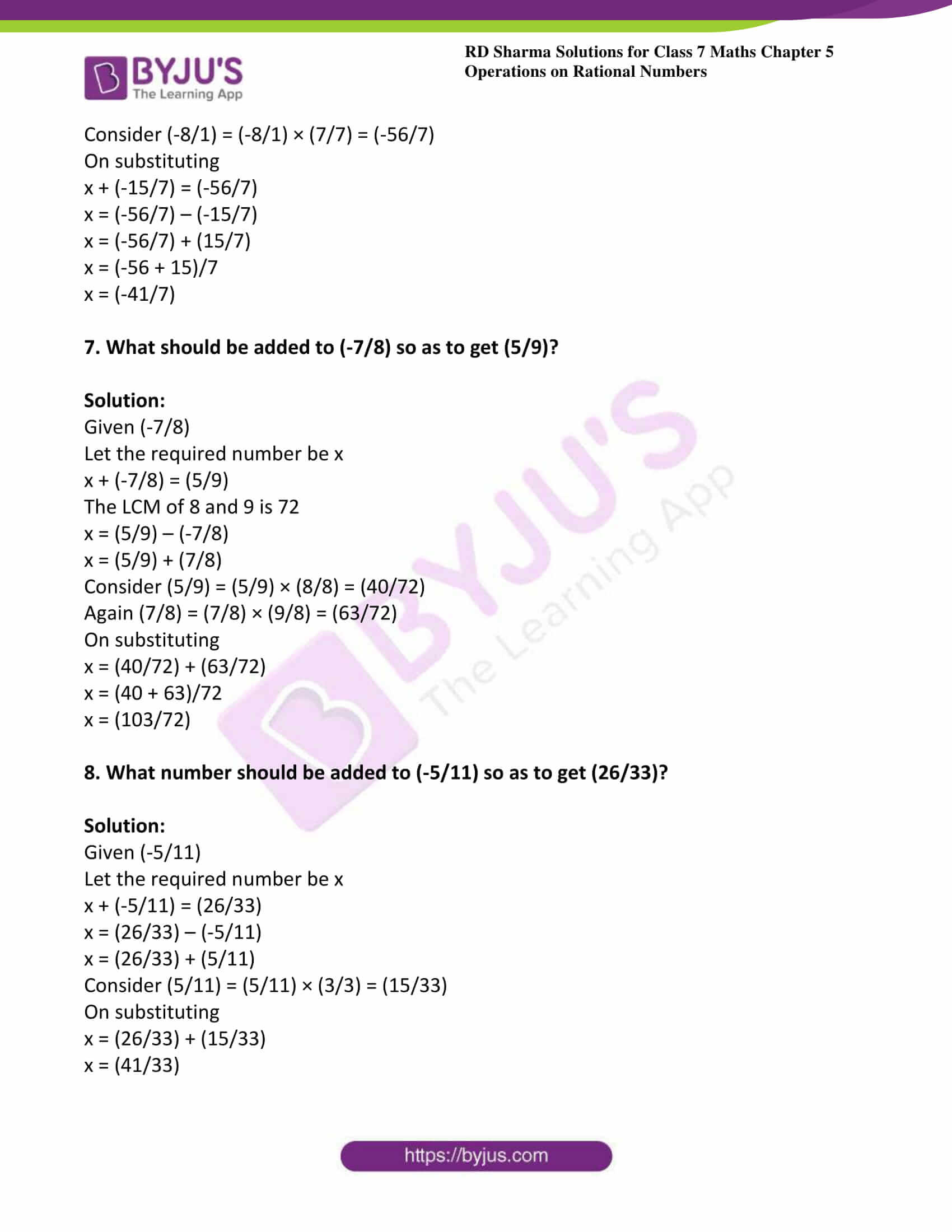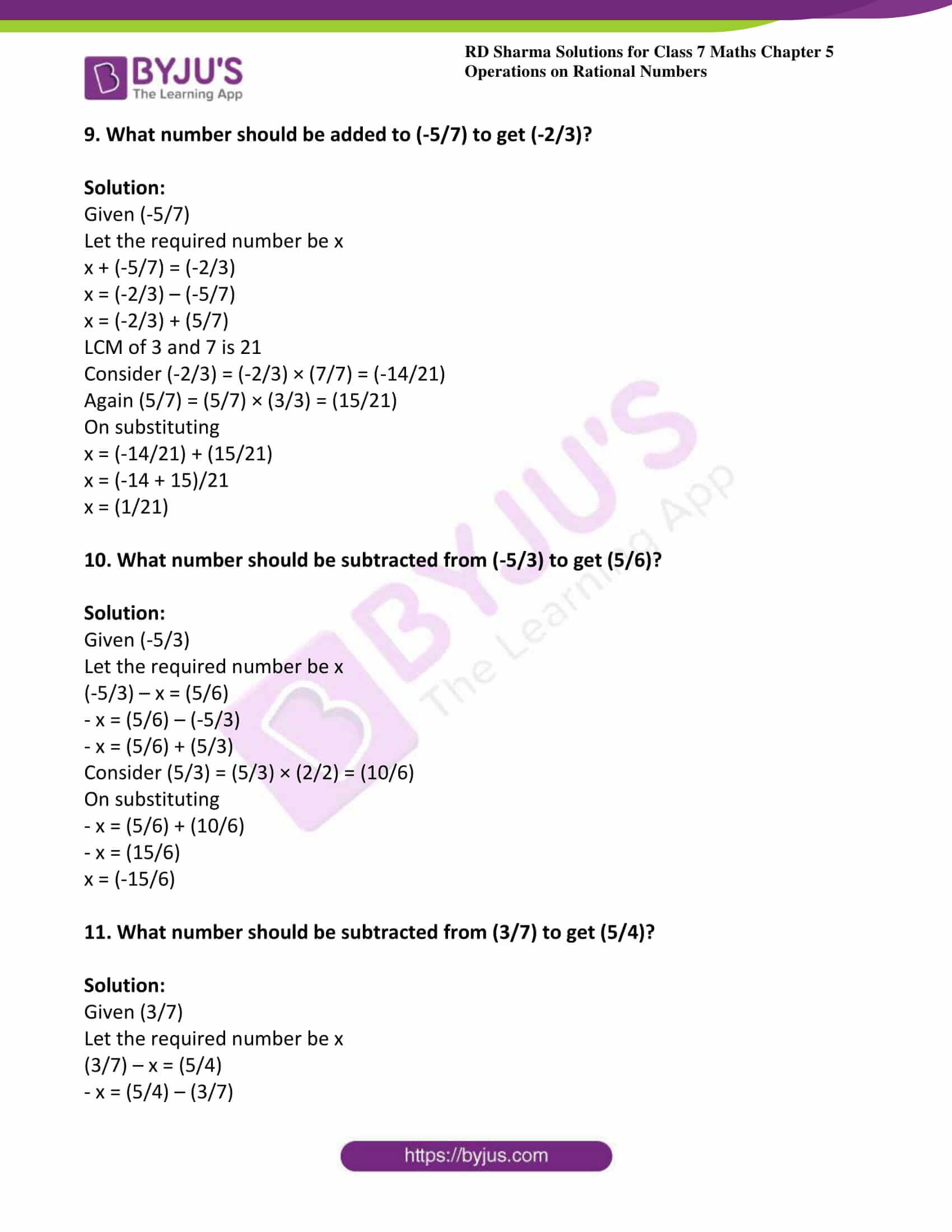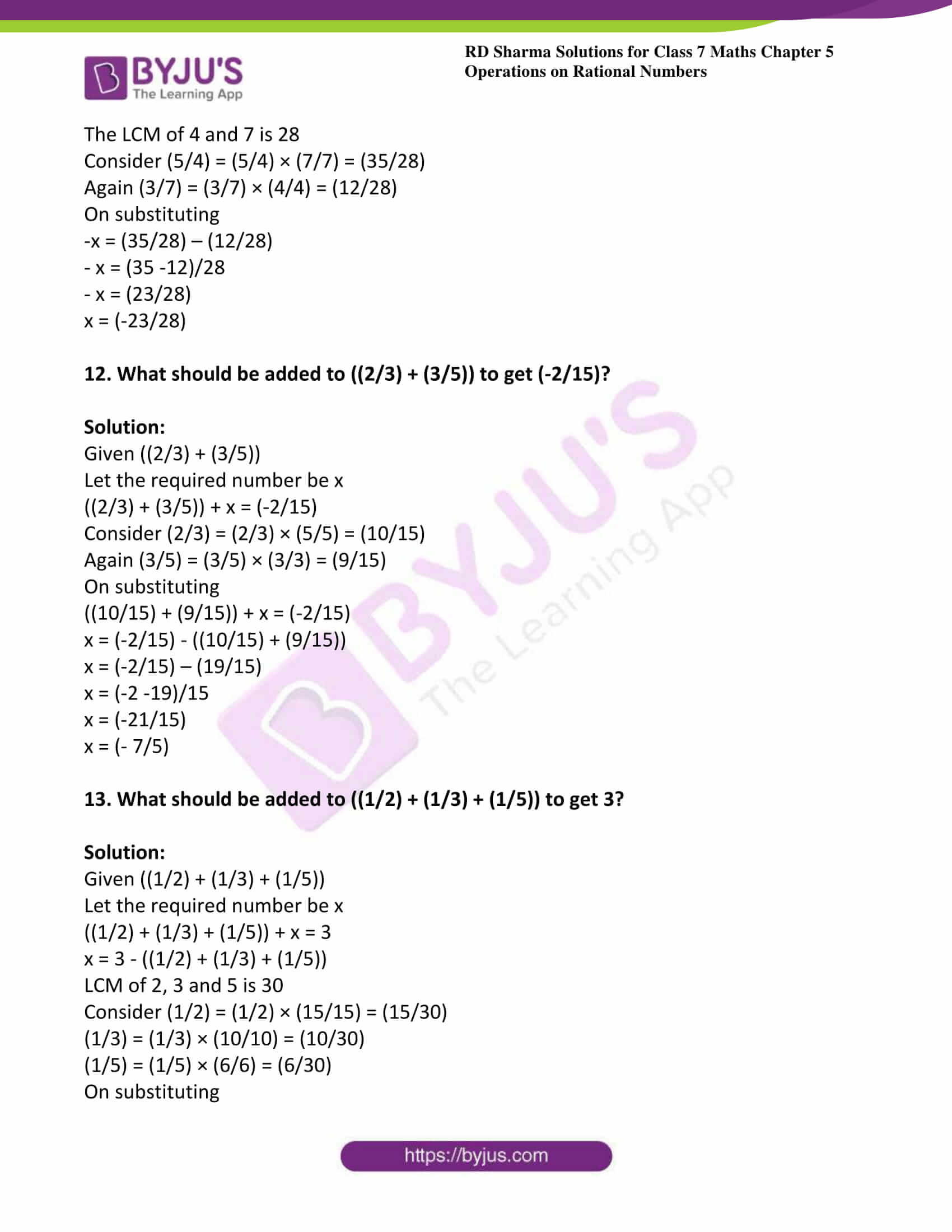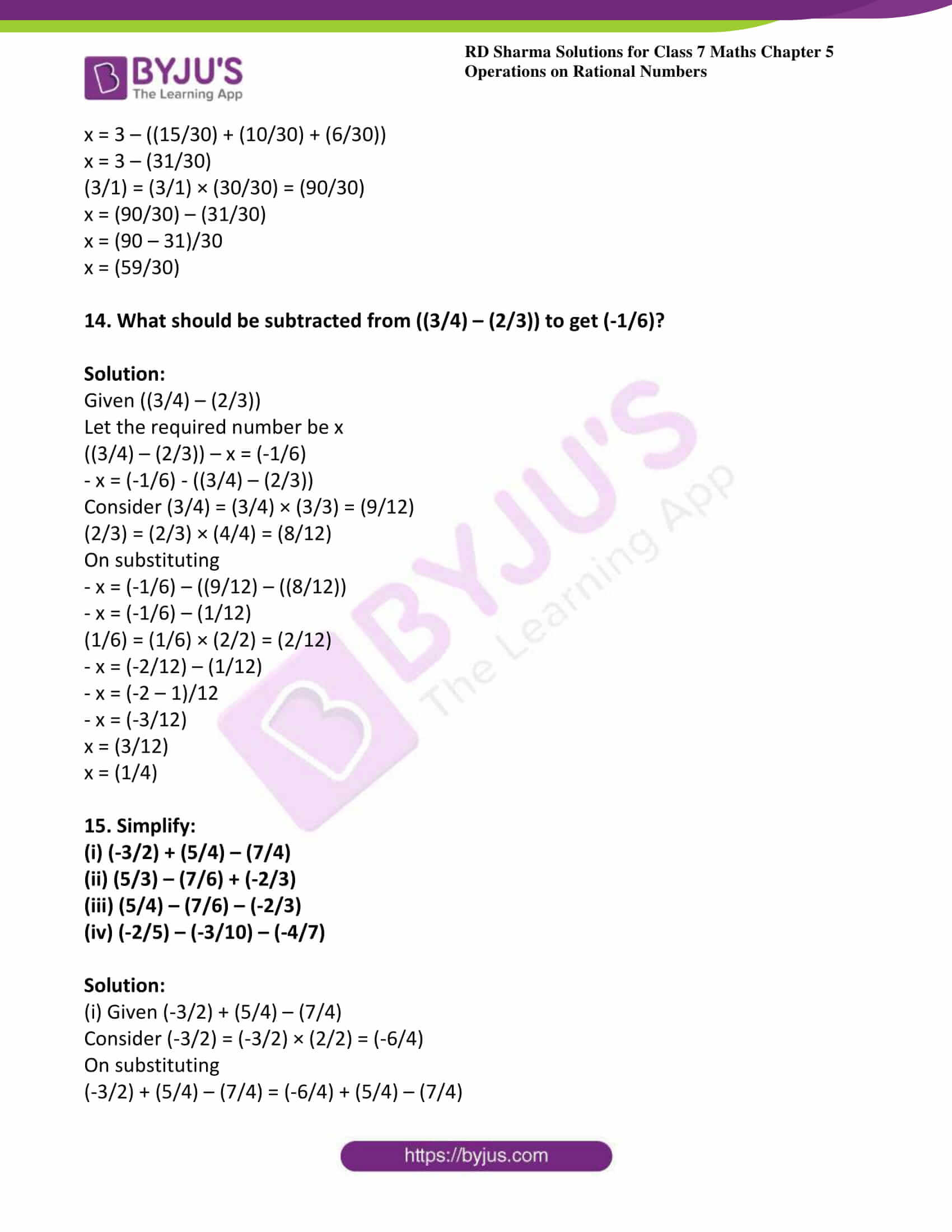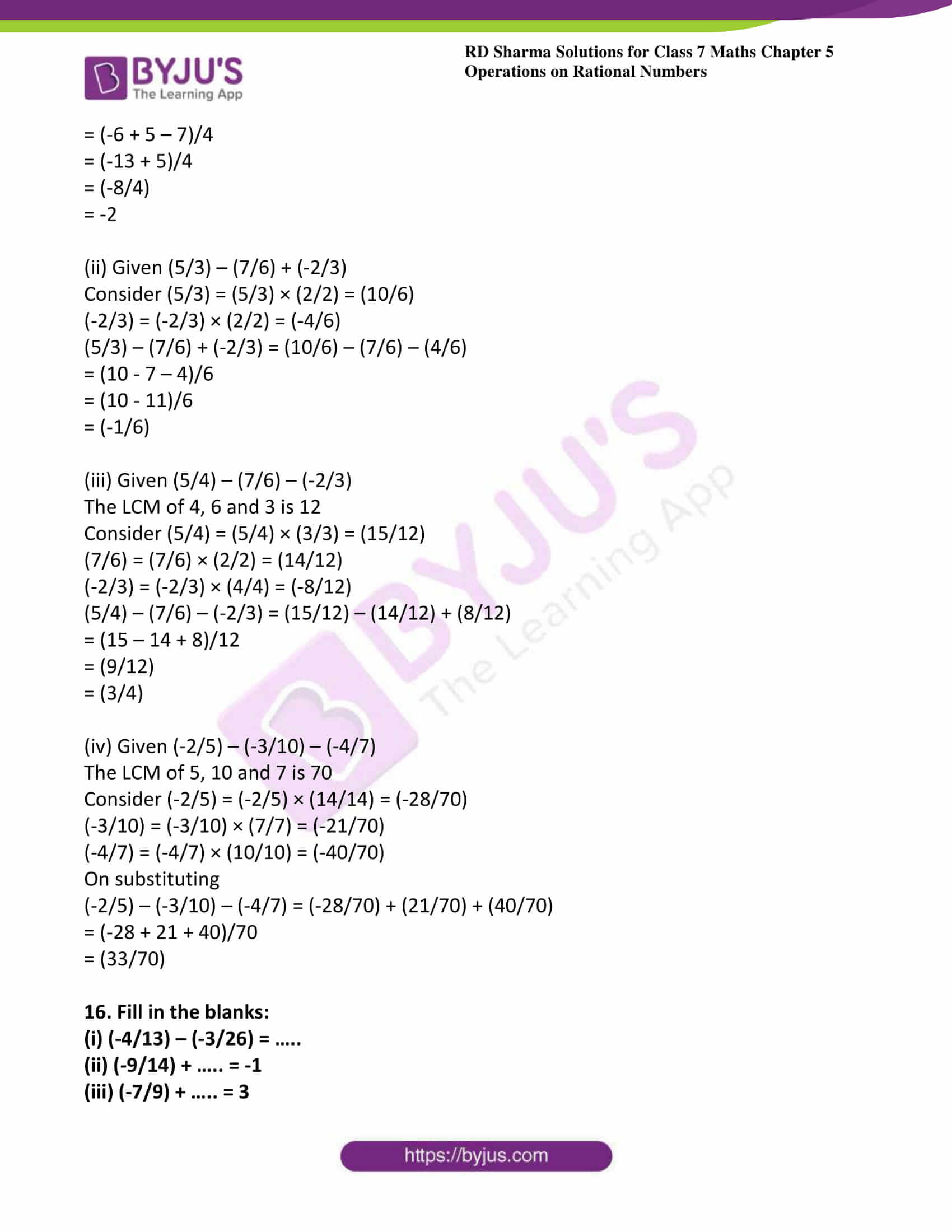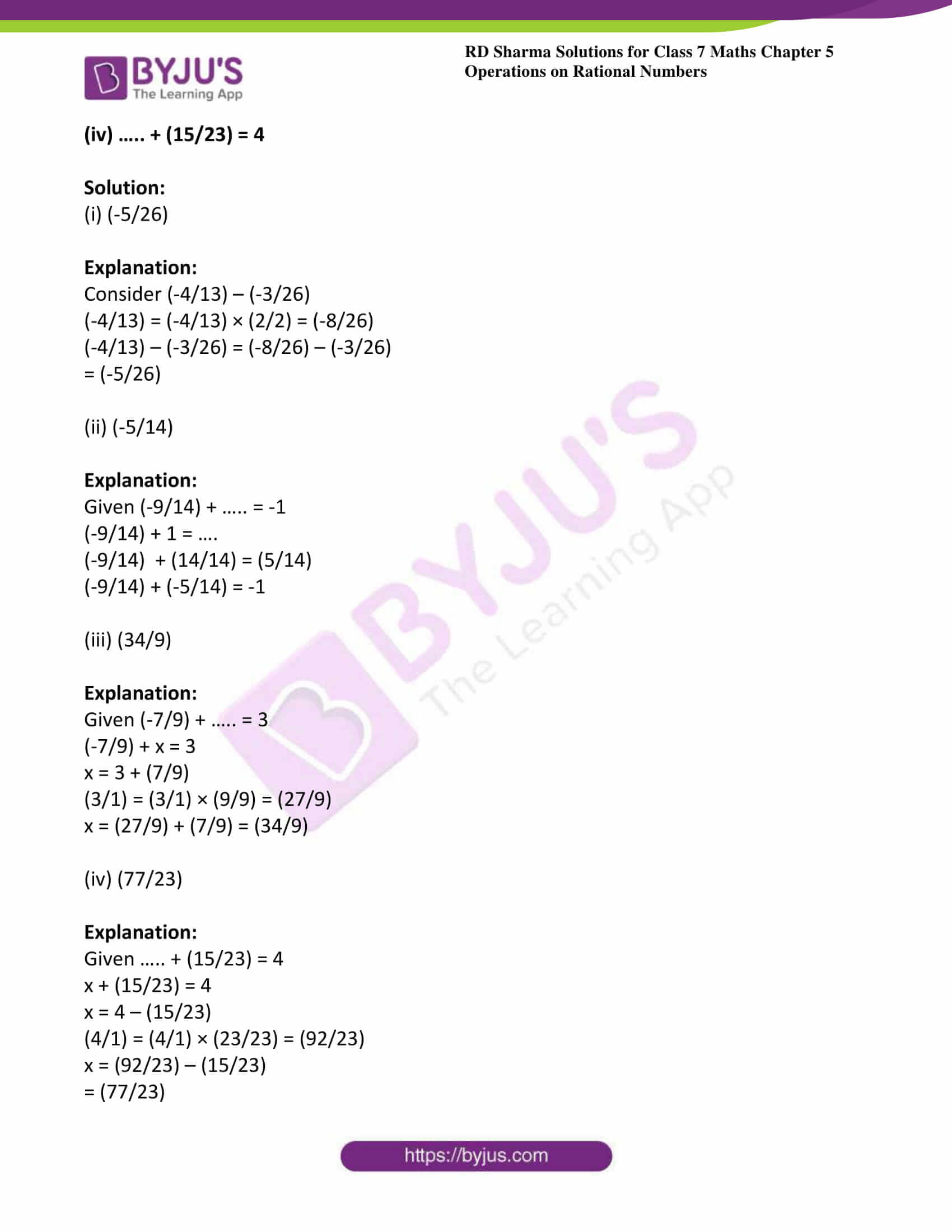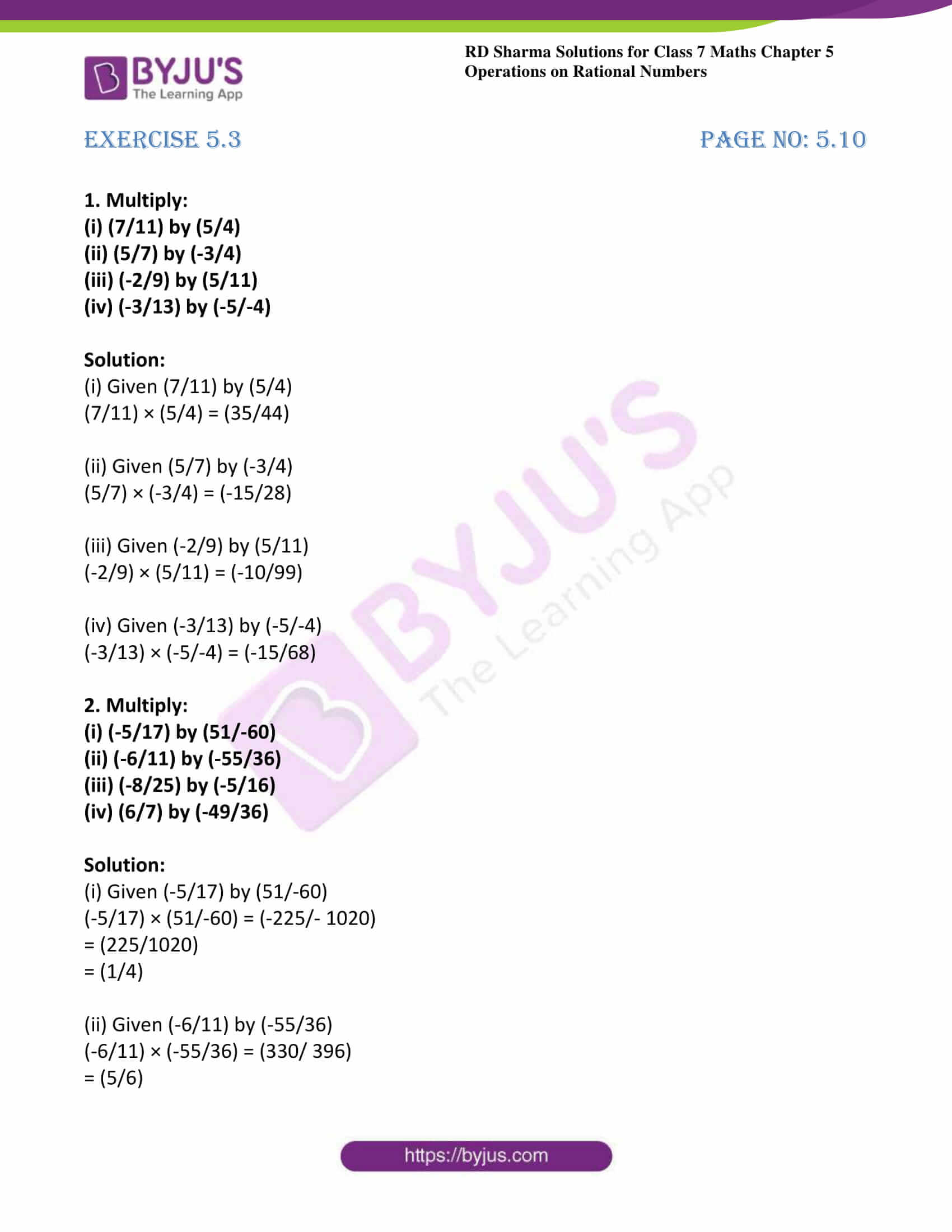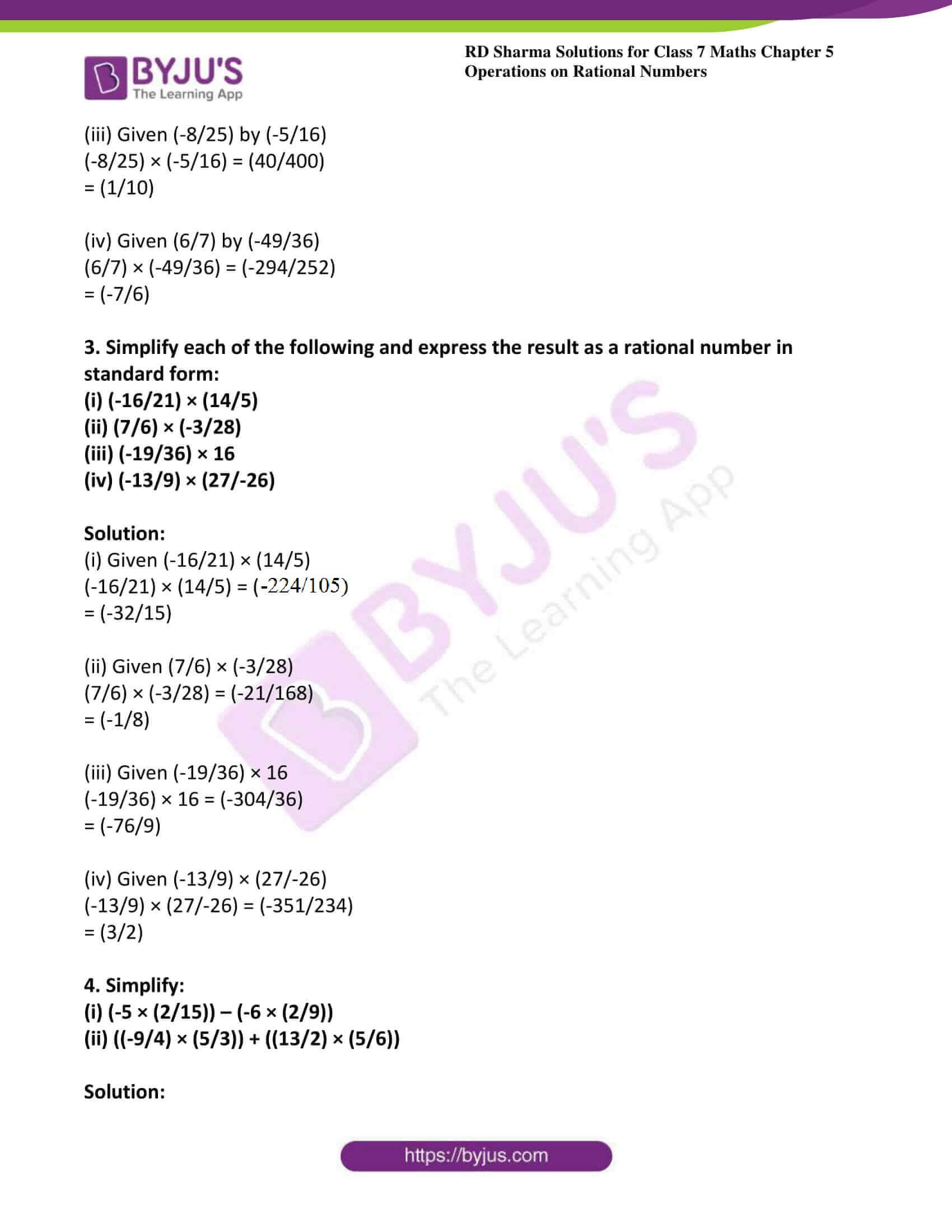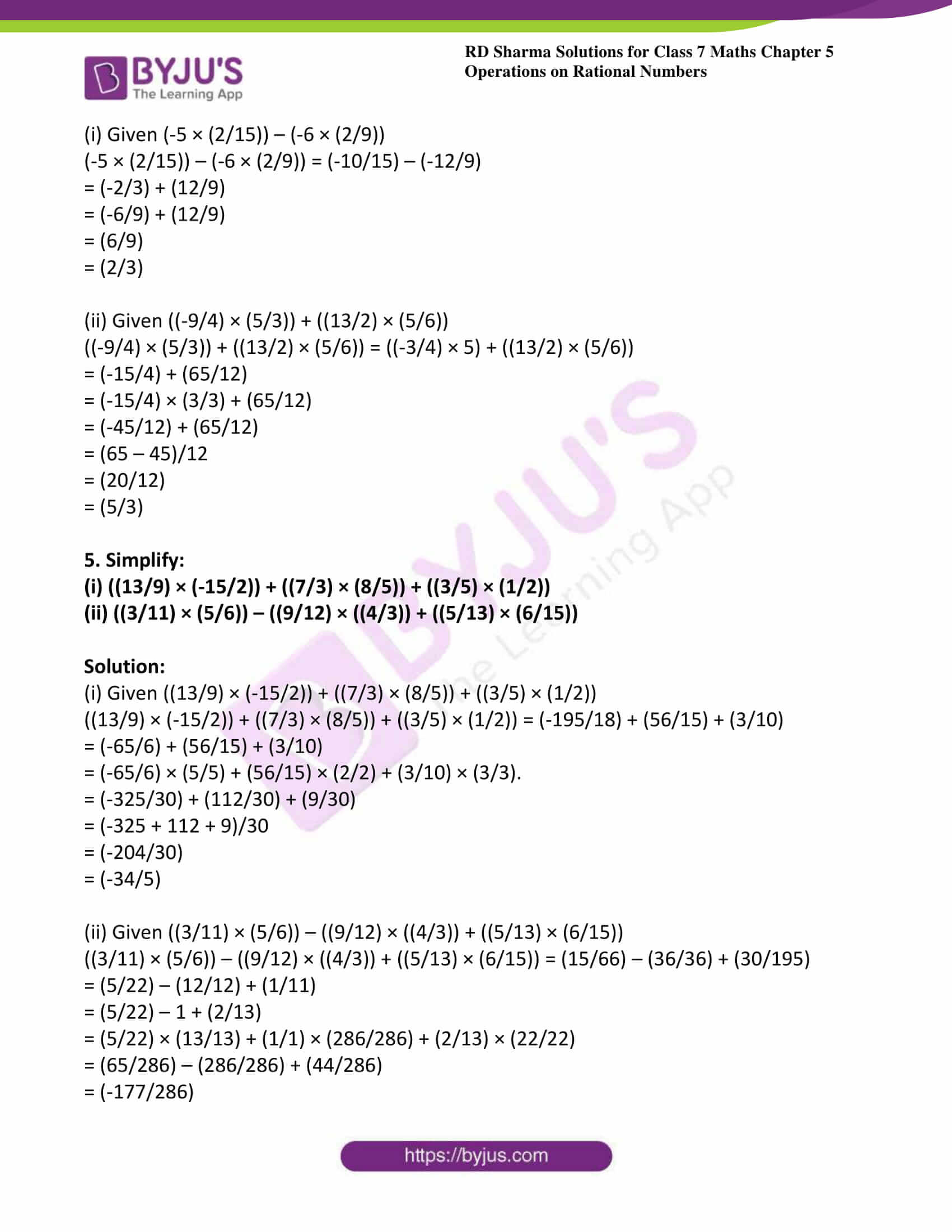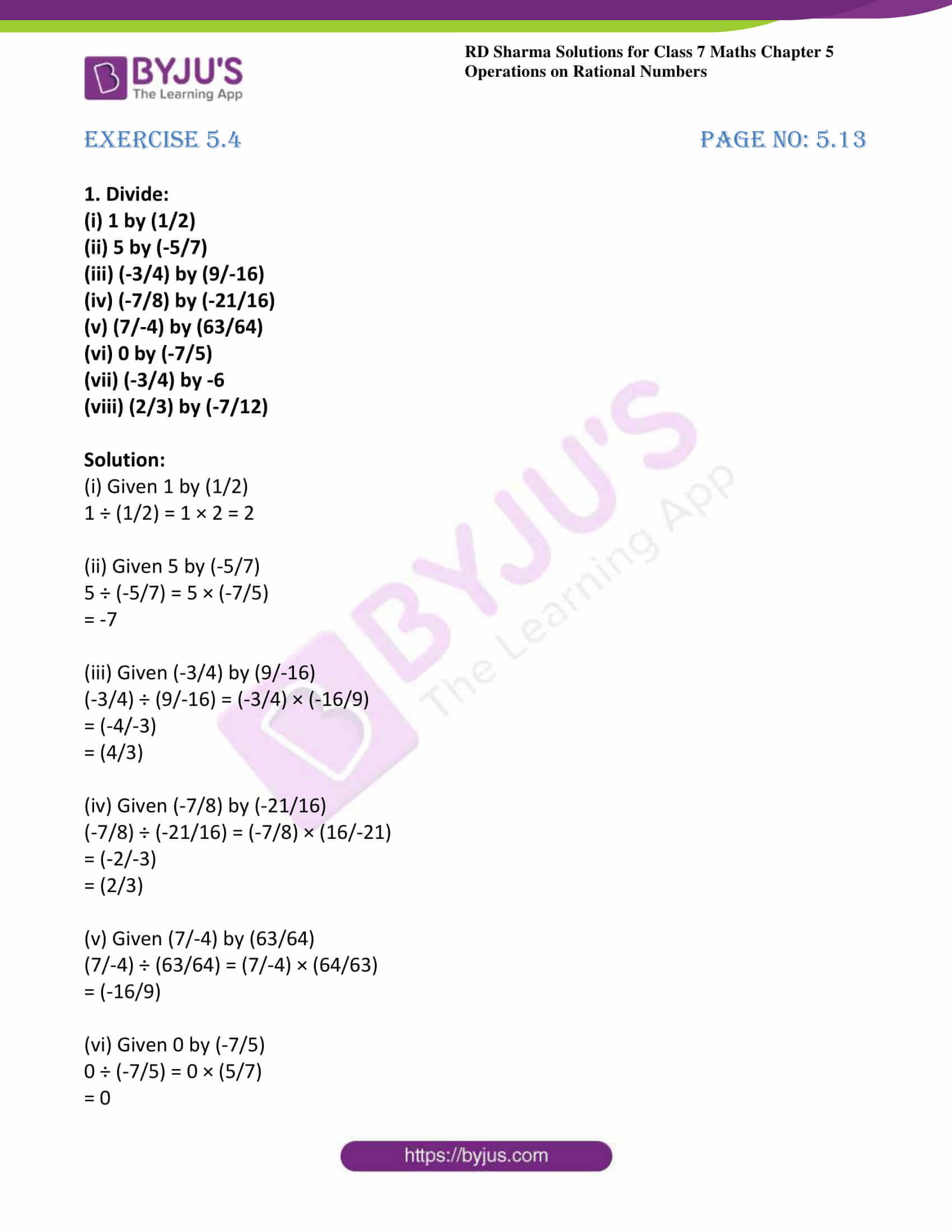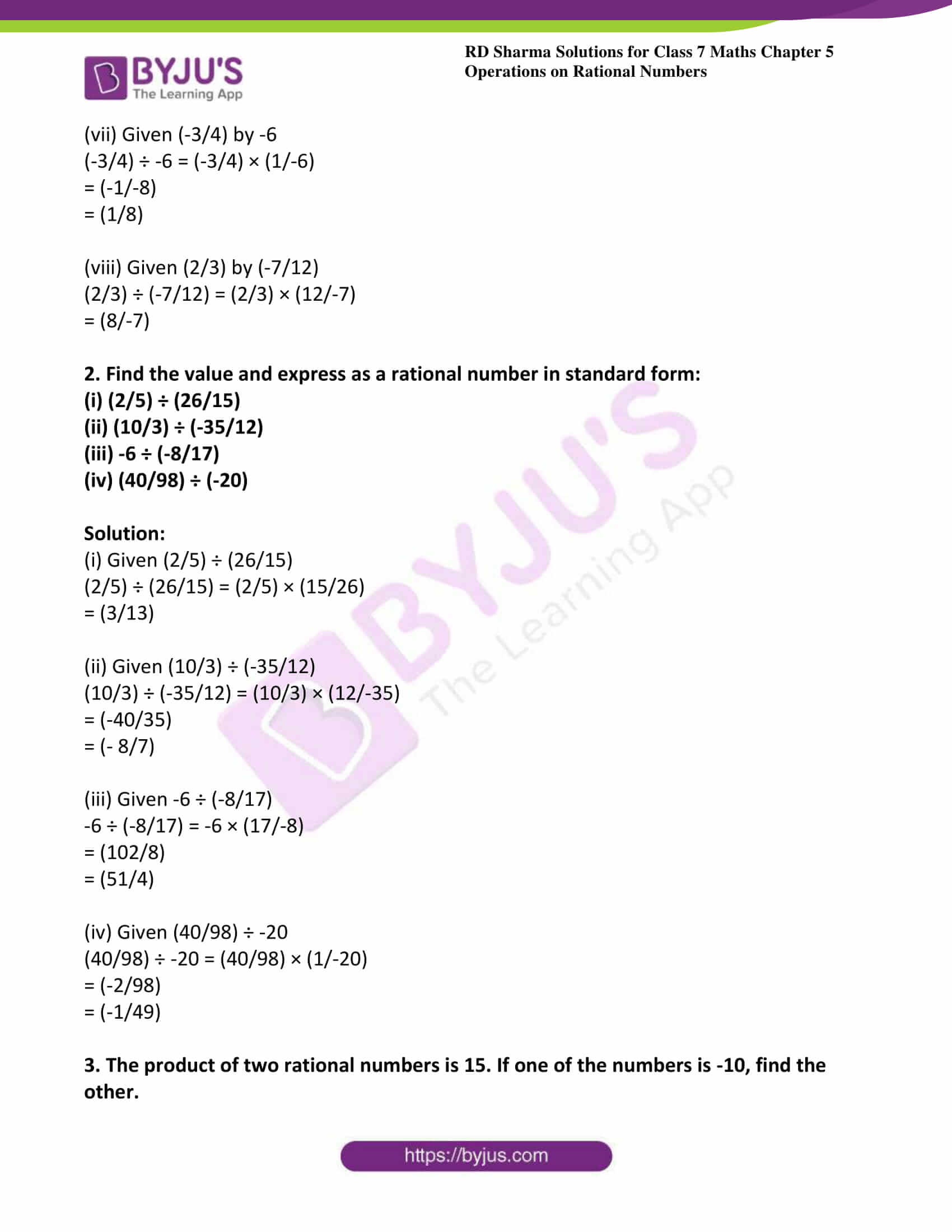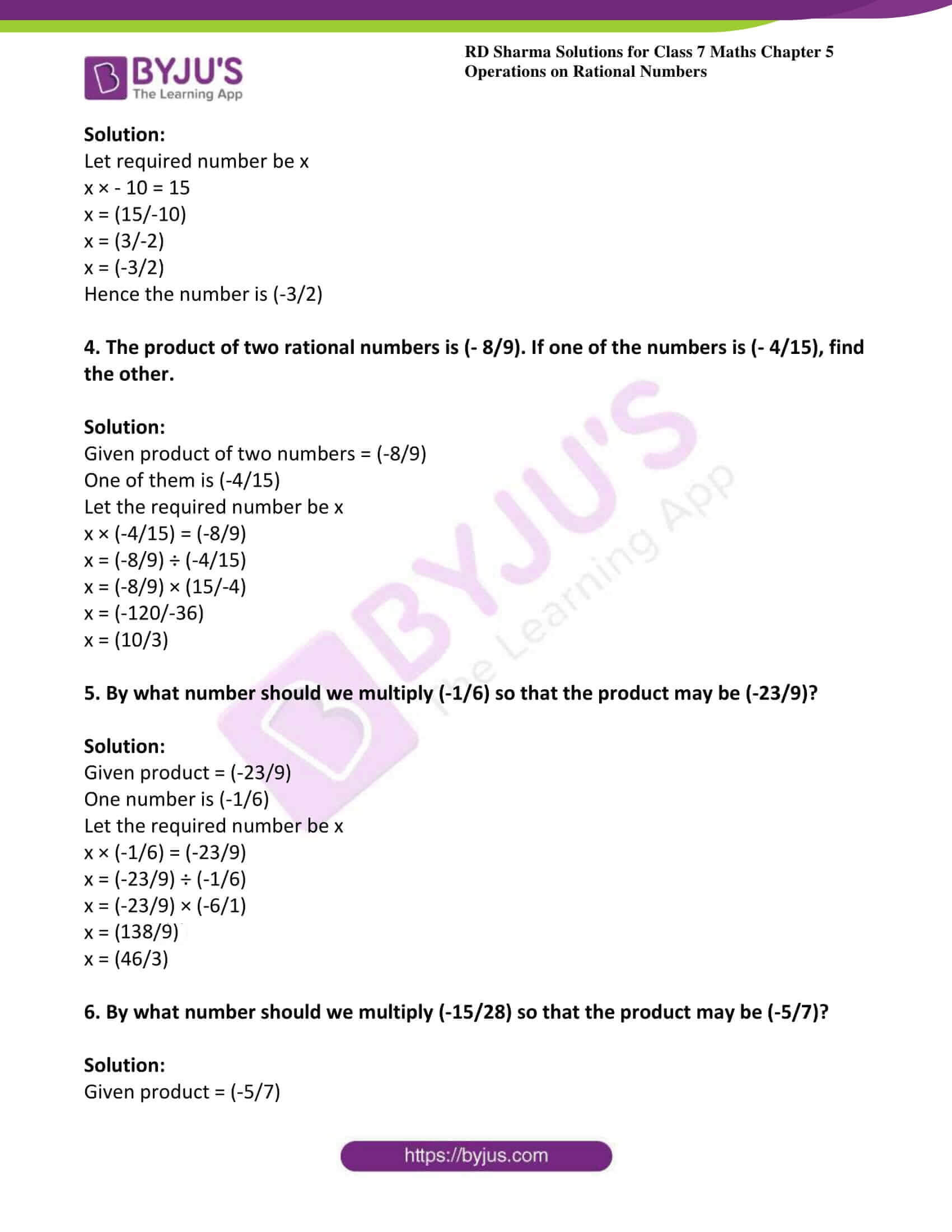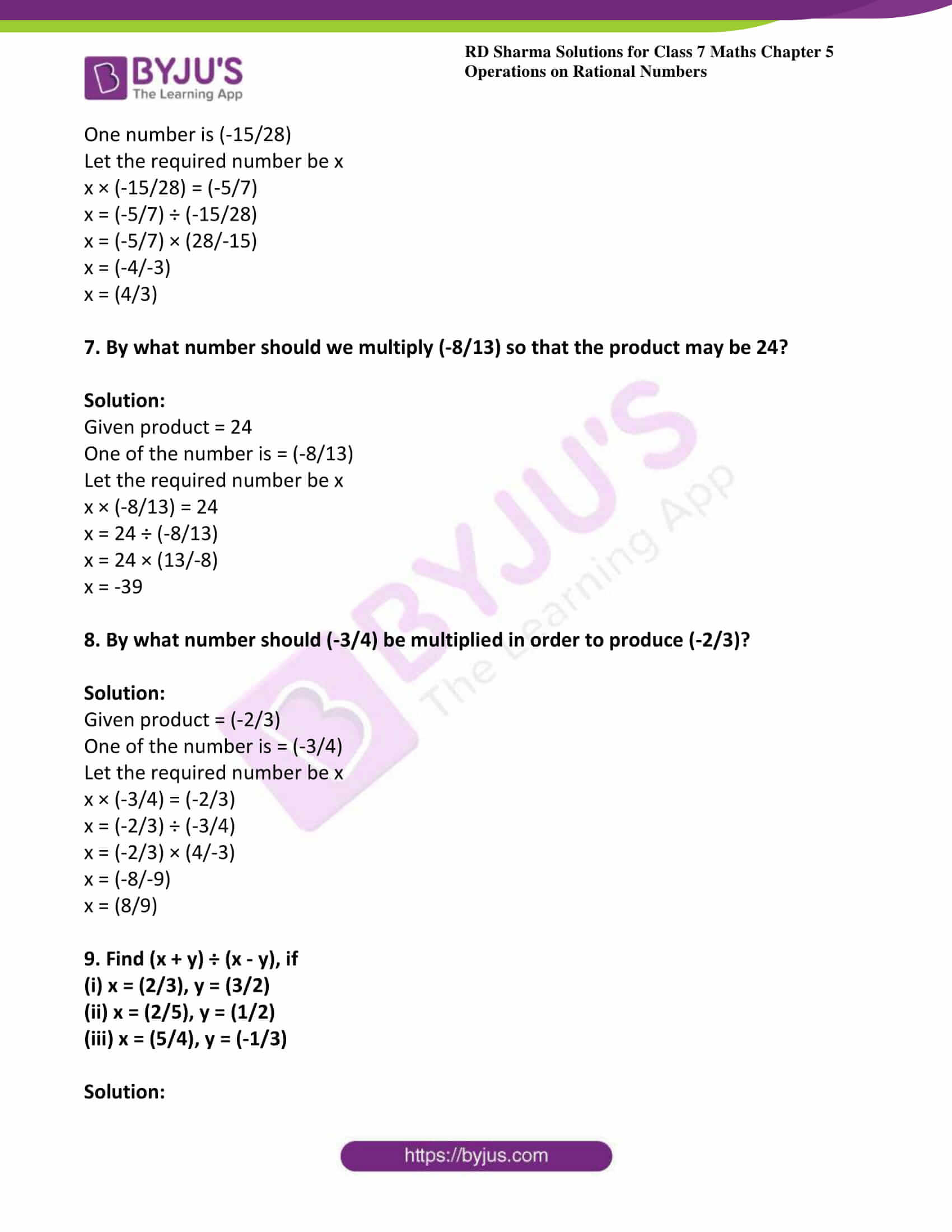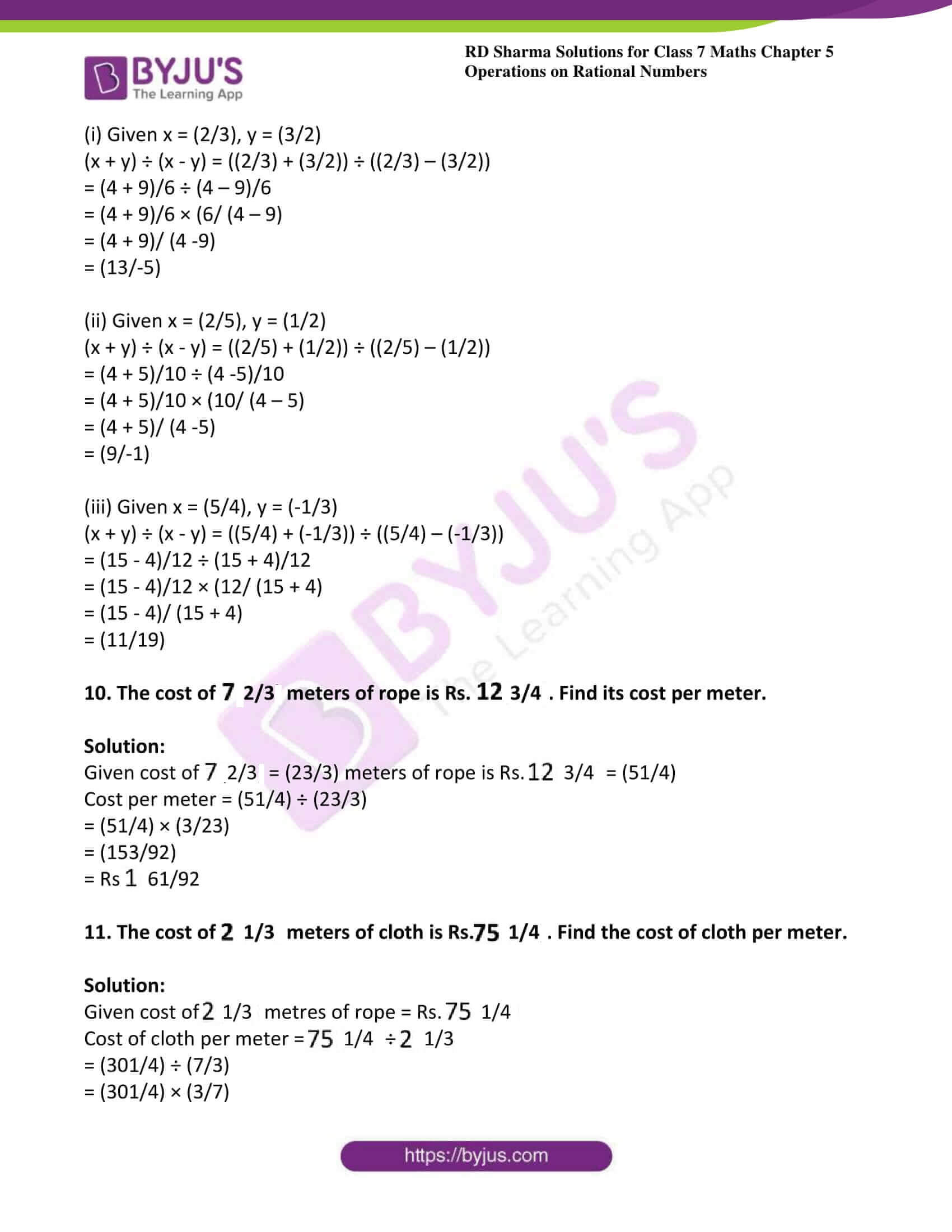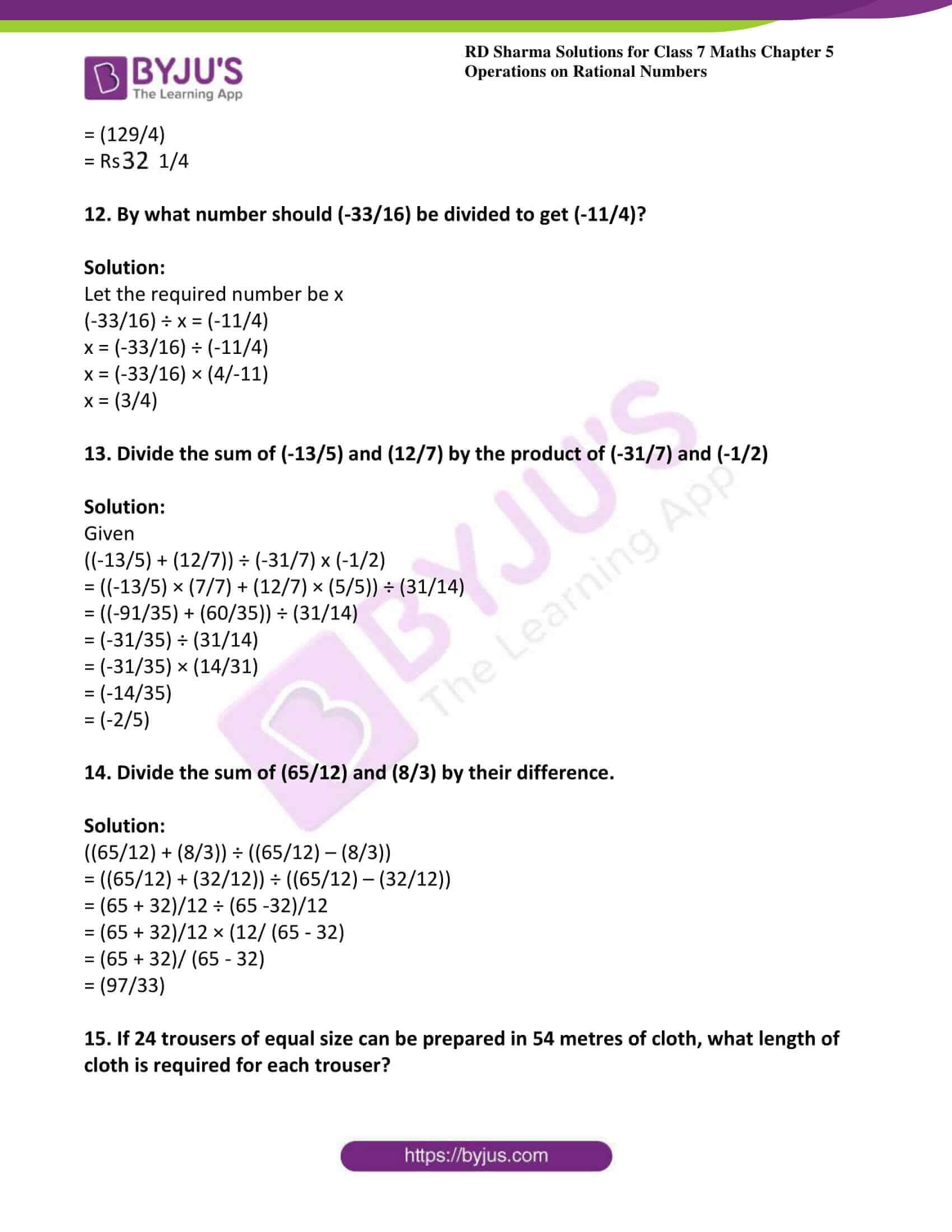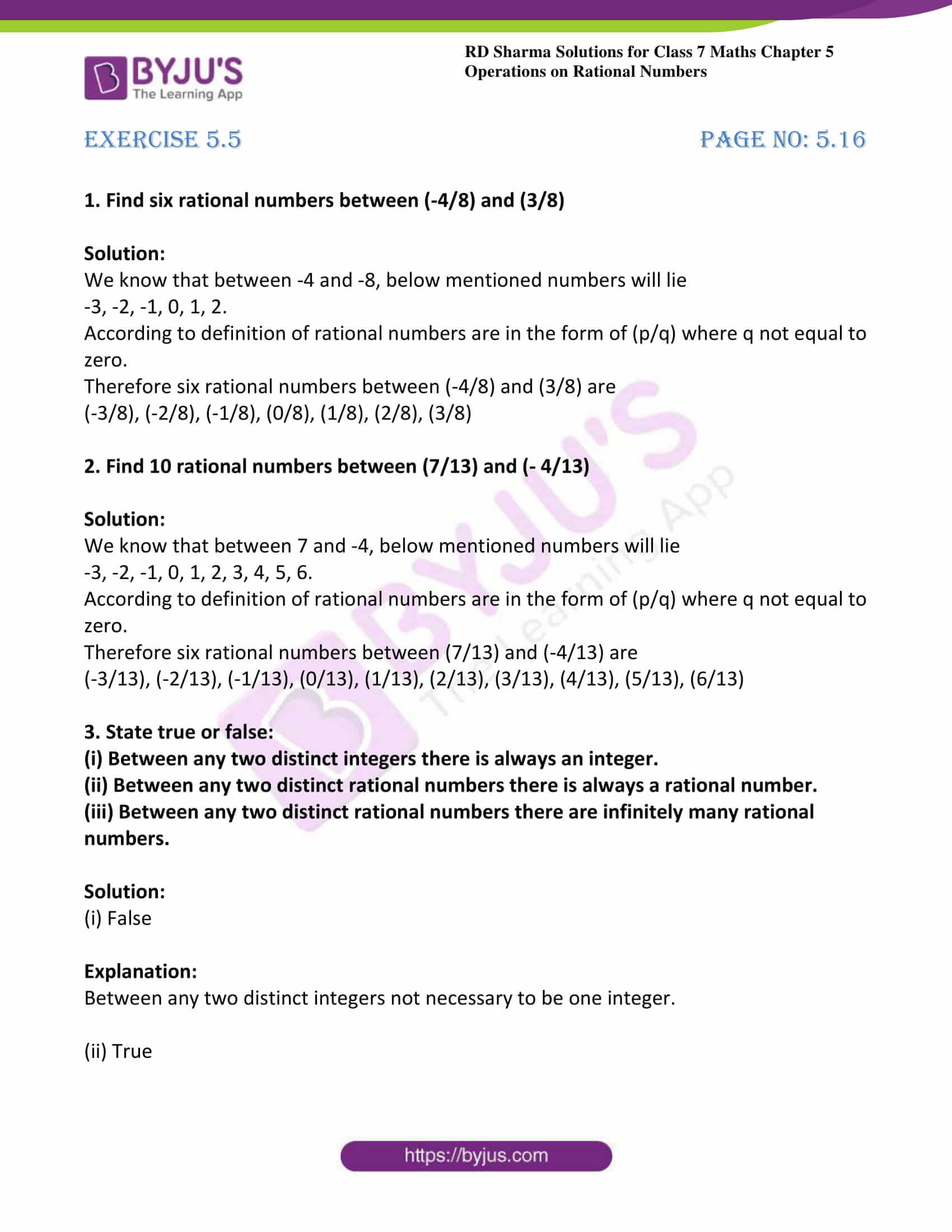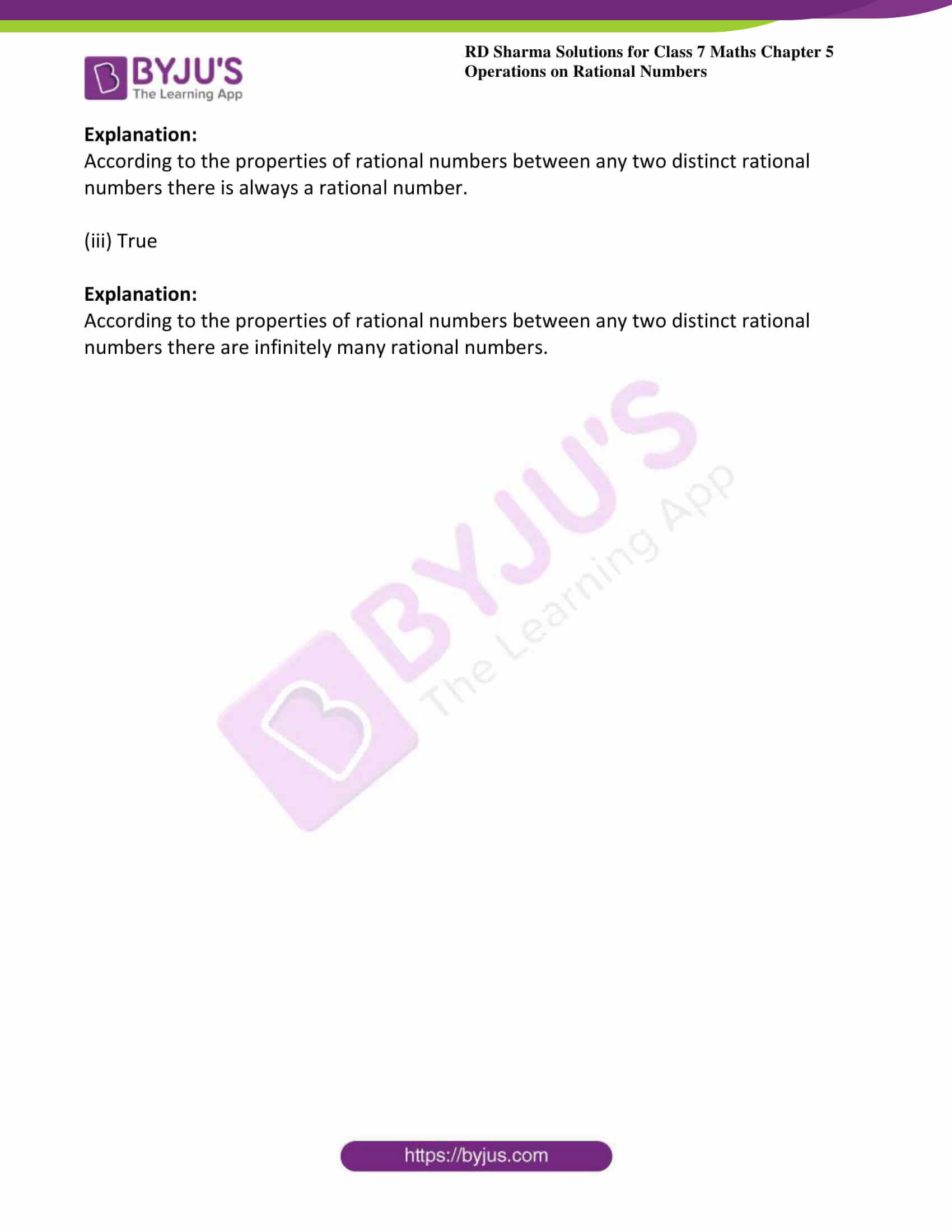## Exercise 5.1 Page No: 5.4

1. Add the following rational numbers:

(i) (-5/7) and (3/7)

(ii) (-15/4) and (7/4)

(iii) (-8/11) and (-4/11)

(iv) (6/13) and (-9/13)

Solution:

(i) Given (-5/7) and (3/7)

= (-5/7) + (3/7)

Here denominators are same so add the numerator

= ((-5+3)/7)

= (-2/7)

(ii) Given (-15/4) and (7/4)

= (-15/4) + (7/4)

Here denominators are same so add the numerator

= ((-15 + 7)/4)

= (-8/4)

On simplifying

= -2

(iii) Given (-8/11) and (-4/11)

= (-8/11) + (-4/11)

Here denominators are same so add the numerator

= (-8 + (-4))/11

= (-12/11)

(iv) Given (6/13) and (-9/13)

= (6/13) + (-9/13)

Here denominators are same so add the numerator

= (6 + (-9))/13

= (-3/13)

2. Add the following rational numbers:

(i) (3/4) and (-3/5)

(ii) -3 and (3/5)

(iii) (-7/27) and (11/18)

(iv) (31/-4) and (-5/8)

Solution:

(i) Given (3/4) and (-3/5)

If p/q and r/s are two rational numbers such that q and s do not have a common factor other than one, then

(p/q) + (r/s) = (p × s + r × q)/ (q × s)

(3/4) + (-3/5) = (3 × 5 + (-3) × 4)/ (4 × 5)

= (15 – 12)/ 20

= (3/20)

(ii) Given -3 and (3/5)

If p/q and r/s are two rational numbers such that q and s do not have a common factor other than one, then

(p/q) + (r/s) = (p × s + r × q)/ (q × s)

(-3/1) + (3/5) = (-3 × 5 + 3 × 1)/ (1 × 5)

= (-15 + 3)/ 5

= (-12/5)

(iii) Given (-7/27) and (11/18)

LCM of 27 and 18 is 54

(-7/27) = (-7/27) × (2/2) = (-14/54)

(11/18) = (11/18) × (3/3) = (33/54)

(-7/27) + (11/18) = (-14 + 33)/54

= (19/54)

(iv) Given (31/-4) and (-5/8)

LCM of -4 and 8 is 8

(31/-4) = (31/-4) × (2/2) = (62/-8)

(31/-4) + (-5/8) = (-62 – 5)/8

= (-67/8)

3. Simplify:

(i) (8/9) + (-11/6)

(ii) (-5/16) + (7/24)

(iii) (1/-12) + (2/-15)

(iv) (-8/19) + (-4/57)

Solution:

(i) Given (8/9) + (-11/6)

The LCM of 9 and 6 is 18

(8/9) = (8/9) × (2/2) = (16/18)

(-11/6) = (-11/6) × (3/3) = (-33/18)

= (16 – 33)/18

= (-17/18)

(ii) Given (-5/16) + (7/24)

The LCM of 16 and 24 is 48

Now (-5/16) = (-5/16) × (3/3) = (-15/48)

Consider (7/24) = (7/24) × (2/2) = (14/48)

(-5/16) + (7/24) = (-15/48) + (14/48)

= (14 – 15) /48

= (-1/48)

(iii) Given (1/-12) + (2/-15)

The LCM of 12 and 15 is 60

Consider (-1/12) = (-1/12) × (5/5) = (-5/60)

Now (2/-15) = (-2/15) × (4/4) = (-8/60)

(1/-12) + (2/-15) = (-5/60) + (-8/60)

= (-5 – 8)/60

= (-13/60)

(iv) Given (-8/19) + (-4/57)

The LCM of 19 and 57 is 57

Consider (-8/57) = (-8/57) × (3/3) = (-24/57)

(-8/19) + (-4/57) = (-24/57) + (-4/57)

= (-24 – 4)/57

= (-28/57)

4. Add and express the sum as mixed fraction:

(i) (-12/5) + (43/10)

(iii) (-31/6) + (-27/8)

Solution:

(i) Given (-12/5) + (43/10)

The LCM of 5 and 10 is 10

Consider (-12/5) = (-12/5) × (2/2) = (-24/10)

(-12/5) + (43/10) = (-24/10) + (43/10)

= (-24 + 43)/10

= (19/10)

Now converting it into mixed fraction

= $1\frac{9}{10}$

The LCM of 7 and 4 is 28

Again (-11/4) = (-11/4) × (7/7) = (-77/28)

(24/7) + (-11/4) = (96/28) + (-77/28)

= (96 – 77)/28

= (19/28)

(iii) Given (-31/6) + (-27/8)

The LCM of 6 and 8 is 24

Consider (-31/6) = (-31/6) × (4/4) = (-124/24)

Again (-27/8) = (-27/8) × (3/3) = (-81/24)

(-31/6) + (-27/8) = (-124/24) + (-81/24)

= (-124 – 81)/24

= (-205/24)

Now converting it into mixed fraction

= $-8\frac{13}{24}$

## Exercise 5.2 Page No: 5.7

1. Subtract the first rational number from the second in each of the following:

(i) (3/8), (5/8)

(ii) (-7/9), (4/9)

(iii) (-2/11), (-9/11)

(iv) (11/13), (-4/13)

Solution:

(i) Given (3/8), (5/8)

(5/8) – (3/8) = (5 – 3)/8

= (2/8)

= (1/4)

(ii) Given (-7/9), (4/9)

(4/9) – (-7/9) = (4/9) + (7/9)

= (4 + 7)/9

= (11/9)

(iii) Given (-2/11), (-9/11)

(-9/11) – (-2/11) = (-9/11) + (2/11)

= (-9 + 2)/ 11

= (-7/11)

(iv) Given (11/13), (-4/13)

(-4/13) – (11/13) = (-4 – 11)/13

= (-15/13)

2. Evaluate each of the following:

(i) (2/3) – (3/5)

(ii) (-4/7) – (2/-3)

(iii) (4/7) – (-5/-7)

(iv) -2 – (5/9)

Solution:

(i) Given (2/3) – (3/5)

The LCM of 3 and 5 is 15

Consider (2/3) = (2/3) × (5/5) = (10/15)

Now again (3/5) = (3/5) × (3/3) = (9/15)

(2/3) – (3/5) = (10/15) – (9/15)

= (1/15)

(ii) Given (-4/7) – (2/-3)

The LCM of 7 and 3 is 21

Consider (-4/7) = (-4/7) × (3/3) = (-12/21)

Again (2/-3) = (-2/3) × (7/7) = (-14/21)

(-4/7) – (2/-3) = (-12/21) – (-14/21)

= (-12 + 14)/21

= (2/21)

(iii) Given (4/7) – (-5/-7)

(4/7) – (5/7) = (4 -5)/7

= (-1/7)

(iv) Given -2 – (5/9)

Consider (-2/1) = (-2/1) × (9/9) = (-18/9)

-2 – (5/9) = (-18/9) – (5/9)

= (-18 -5)/9

= (-23/9)

3. The sum of the two numbers is (5/9). If one of the numbers is (1/3), find the other.

Solution:

Given sum of two numbers is (5/9)

And one them is (1/3)

Let the unknown number be x

x + (1/3) = (5/9)

x = (5/9) – (1/3)

LCM of 3 and 9 is 9

Consider (1/3) = (1/3) × (3/3) = (3/9)

On substituting we get

x = (5/9) – (3/9)

x = (5 – 3)/9

x = (2/9)

4. The sum of two numbers is (-1/3). If one of the numbers is (-12/3), find the other.

Solution:

Given sum of two numbers = (-1/3)

One of them is (-12/3)

Let the required number be x

x + (-12/3) = (-1/3)

x = (-1/3) – (-12/3)

x = (-1/3) + (12/3)

x = (-1 + 12)/3

x = (11/3)

5. The sum of two numbers is (– 4/3). If one of the numbers is -5, find the other.

Solution:

Given sum of two numbers = (-4/3)

One of them is -5

Let the required number be x

x + (-5) = (-4/3)

LCM of 1 and 3 is 3

(-5/1) = (-5/1) × (3/3) = (-15/3)

On substituting

x + (-15/3) = (-4/3)

x = (-4/3) – (-15/3)

x = (-4/3) + (15/3)

x = (-4 + 15)/3

x = (11/3)

6. The sum of two rational numbers is – 8. If one of the numbers is (-15/7), find the other.

Solution:

Given sum of two numbers is -8

One of them is (-15/7)

Let the required number be x

x + (-15/7) = -8

The LCM of 7 and 1 is 7

Consider (-8/1) = (-8/1) × (7/7) = (-56/7)

On substituting

x + (-15/7) = (-56/7)

x = (-56/7) – (-15/7)

x = (-56/7) + (15/7)

x = (-56 + 15)/7

x = (-41/7)

7. What should be added to (-7/8) so as to get (5/9)?

Solution:

Given (-7/8)

Let the required number be x

x + (-7/8) = (5/9)

The LCM of 8 and 9 is 72

x = (5/9) – (-7/8)

x = (5/9) + (7/8)

Consider (5/9) = (5/9) × (8/8) = (40/72)

Again (7/8) = (7/8) × (9/8) = (63/72)

On substituting

x = (40/72) + (63/72)

x = (40 + 63)/72

x = (103/72)

8. What number should be added to (-5/11) so as to get (26/33)?

Solution:

Given (-5/11)

Let the required number be x

x + (-5/11) = (26/33)

x = (26/33) – (-5/11)

x = (26/33) + (5/11)

Consider (5/11) = (5/11) × (3/3) = (15/33)

On substituting

x = (26/33) + (15/33)

x = (41/33)

9. What number should be added to (-5/7) to get (-2/3)?

Solution:

Given (-5/7)

Let the required number be x

x + (-5/7) = (-2/3)

x = (-2/3) – (-5/7)

x = (-2/3) + (5/7)

LCM of 3 and 7 is 21

Consider (-2/3) = (-2/3) × (7/7) = (-14/21)

Again (5/7) = (5/7) × (3/3) = (15/21)

On substituting

x = (-14/21) + (15/21)

x = (-14 + 15)/21

x = (1/21)

10. What number should be subtracted from (-5/3) to get (5/6)?

Solution:

Given (-5/3)

Let the required number be x

(-5/3) – x = (5/6)

– x = (5/6) – (-5/3)

– x = (5/6) + (5/3)

Consider (5/3) = (5/3) × (2/2) = (10/6)

On substituting

– x = (5/6) + (10/6)

– x = (15/6)

x = (-15/6)

11. What number should be subtracted from (3/7) to get (5/4)?

Solution:

Given (3/7)

Let the required number be x

(3/7) – x = (5/4)

– x = (5/4) – (3/7)

The LCM of 4 and 7 is 28

Consider (5/4) = (5/4) × (7/7) = (35/28)

Again (3/7) = (3/7) × (4/4) = (12/28)

On substituting

-x = (35/28) – (12/28)

– x = (35 -12)/28

– x = (23/28)

x = (-23/28)

12. What should be added to ((2/3) + (3/5)) to get (-2/15)?

Solution:

Given ((2/3) + (3/5))

Let the required number be x

((2/3) + (3/5)) + x = (-2/15)

Consider (2/3) = (2/3) × (5/5) = (10/15)

Again (3/5) = (3/5) × (3/3) = (9/15)

On substituting

((10/15) + (9/15)) + x = (-2/15)

x = (-2/15) – ((10/15) + (9/15))

x = (-2/15) – (19/15)

x = (-2 -19)/15

x = (-21/15)

x = (- 7/5)

13. What should be added to ((1/2) + (1/3) + (1/5)) to get 3?

Solution:

Given ((1/2) + (1/3) + (1/5))

Let the required number be x

((1/2) + (1/3) + (1/5)) + x = 3

x = 3 – ((1/2) + (1/3) + (1/5))

LCM of 2, 3 and 5 is 30

Consider (1/2) = (1/2) × (15/15) = (15/30)

(1/3) = (1/3) × (10/10) = (10/30)

(1/5) = (1/5) × (6/6) = (6/30)

On substituting

x = 3 – ((15/30) + (10/30) + (6/30))

x = 3 – (31/30)

(3/1) = (3/1) × (30/30) = (90/30)

x = (90/30) – (31/30)

x = (90 – 31)/30

x = (59/30)

14. What should be subtracted from ((3/4) – (2/3)) to get (-1/6)?

Solution:

Given ((3/4) – (2/3))

Let the required number be x

((3/4) – (2/3)) – x = (-1/6)

– x = (-1/6) – ((3/4) – (2/3))

Consider (3/4) = (3/4) × (3/3) = (9/12)

(2/3) = (2/3) × (4/4) = (8/12)

On substituting

– x = (-1/6) – ((9/12) – ((8/12))

– x = (-1/6) – (1/12)

(1/6) = (1/6) × (2/2) = (2/12)

– x = (-2/12) – (1/12)

– x = (-2 – 1)/12

– x = (-3/12)

x = (3/12)

x = (1/4)

15. Simplify:

(i) (-3/2) + (5/4) – (7/4)

(ii) (5/3) – (7/6) + (-2/3)

(iii) (5/4) – (7/6) – (-2/3)

(iv) (-2/5) – (-3/10) – (-4/7)

Solution:

(i) Given (-3/2) + (5/4) – (7/4)

Consider (-3/2) = (-3/2) × (2/2) = (-6/4)

On substituting

(-3/2) + (5/4) – (7/4) = (-6/4) + (5/4) – (7/4)

= (-6 + 5 – 7)/4

= (-13 + 5)/4

= (-8/4)

= -2

(ii) Given (5/3) – (7/6) + (-2/3)

Consider (5/3) = (5/3) × (2/2) = (10/6)

(-2/3) = (-2/3) × (2/2) = (-4/6)

(5/3) – (7/6) + (-2/3) = (10/6) – (7/6) – (4/6)

= (10 – 7 – 4)/6

= (10 – 11)/6

= (-1/6)

(iii) Given (5/4) – (7/6) – (-2/3)

The LCM of 4, 6 and 3 is 12

Consider (5/4) = (5/4) × (3/3) = (15/12)

(7/6) = (7/6) × (2/2) = (14/12)

(-2/3) = (-2/3) × (4/4) = (-8/12)

(5/4) – (7/6) – (-2/3) = (15/12) – (14/12) + (8/12)

= (15 – 14 + 8)/12

= (9/12)

= (3/4)

(iv) Given (-2/5) – (-3/10) – (-4/7)

The LCM of 5, 10 and 7 is 70

Consider (-2/5) = (-2/5) × (14/14) = (-28/70)

(-3/10) = (-3/10) × (7/7) = (-21/70)

(-4/7) = (-4/7) × (10/10) = (-40/70)

On substituting

(-2/5) – (-3/10) – (-4/7) = (-28/70) + (21/70) + (40/70)

= (-28 + 21 + 40)/70

= (33/70)

16. Fill in the blanks:

(i) (-4/13) – (-3/26) = …..

(ii) (-9/14) + ….. = -1

(iii) (-7/9) + ….. = 3

(iv) ….. + (15/23) = 4

Solution:

(i) (-5/26)

Explanation:

Consider (-4/13) – (-3/26)

(-4/13) = (-4/13) × (2/2) = (-8/26)

(-4/13) – (-3/26) = (-8/26) – (-3/26)

= (-5/26)

(ii) (-5/14)

Explanation:

Given (-9/14) + ….. = -1

(-9/14) + 1 = ….

(-9/14) + (14/14) = (5/14)

(-9/14) + (-5/14) = -1

(iii) (34/9)

Explanation:

Given (-7/9) + ….. = 3

(-7/9) + x = 3

x = 3 + (7/9)

(3/1) = (3/1) × (9/9) = (27/9)

x = (27/9) + (7/9) = (34/9)

(iv) (77/23)

Explanation:

Given ….. + (15/23) = 4

x + (15/23) = 4

x = 4 – (15/23)

(4/1) = (4/1) × (23/23) = (92/23)

x = (92/23) – (15/23)

= (77/23)

## Exercise 5.3 Page No: 5.10

1. Multiply:

(i) (7/11) by (5/4)

(ii) (5/7) by (-3/4)

(iii) (-2/9) by (5/11)

(iv) (-3/13) by (-5/-4)

Solution:

(i) Given (7/11) by (5/4)

(7/11) × (5/4) = (35/44)

(ii) Given (5/7) by (-3/4)

(5/7) × (-3/4) = (-15/28)

(iii) Given (-2/9) by (5/11)

(-2/9) × (5/11) = (-10/99)

(iv) Given (-3/13) by (-5/-4)

(-3/13) × (-5/-4) = (-15/68)

2. Multiply:

(i) (-5/17) by (51/-60)

(ii) (-6/11) by (-55/36)

(iii) (-8/25) by (-5/16)

(iv) (6/7) by (-49/36)

Solution:

(i) Given (-5/17) by (51/-60)

(-5/17) × (51/-60) = (-225/- 1020)

= (225/1020)

= (1/4)

(ii) Given (-6/11) by (-55/36)

(-6/11) × (-55/36) = (330/ 396)

= (5/6)

(iii) Given (-8/25) by (-5/16)

(-8/25) × (-5/16) = (40/400)

= (1/10)

(iv) Given (6/7) by (-49/36)

(6/7) × (-49/36) = (-294/252)

= (-7/6)

3. Simplify each of the following and express the result as a rational number in standard form:

(i) (-16/21) × (14/5)

(ii) (7/6) × (-3/28)

(iii) (-19/36) × 16

(iv) (-13/9) × (27/-26)

Solution:

(i) Given (-16/21) × (14/5)

(-16/21) × (14/5) = (-224/105)

= (-32/15)

(ii) Given (7/6) × (-3/28)

(7/6) × (-3/28) = (-21/168)

= (-1/8)

(iii) Given (-19/36) × 16

(-19/36) × 16 = (-304/36)

= (-76/9)

(iv) Given (-13/9) × (27/-26)

(-13/9) × (27/-26) = (-351/234)

= (3/2)

4. Simplify:

(i) (-5 × (2/15)) – (-6 × (2/9))

(ii) ((-9/4) × (5/3)) + ((13/2) × (5/6))

Solution:

(i) Given (-5 × (2/15)) – (-6 × (2/9))

(-5 × (2/15)) – (-6 × (2/9)) = (-10/15) – (-12/9)

= (-2/3) + (12/9)

= (-6/9) + (12/9)

= (6/9)

= (2/3)

(ii) Given ((-9/4) × (5/3)) + ((13/2) × (5/6))

((-9/4) × (5/3)) + ((13/2) × (5/6)) = ((-3/4) × 5) + ((13/2) × (5/6))

= (-15/4) + (65/12)

= (-15/4) × (3/3) + (65/12)

= (-45/12) + (65/12)

= (65 – 45)/12

= (20/12)

= (5/3)

5. Simplify:

(i) ((13/9) × (-15/2)) + ((7/3) × (8/5)) + ((3/5) × (1/2))

(ii) ((3/11) × (5/6)) – ((9/12) × ((4/3)) + ((5/13) × (6/15))

Solution:

(i) Given ((13/9) × (-15/2)) + ((7/3) × (8/5)) + ((3/5) × (1/2))

((13/9) × (-15/2)) + ((7/3) × (8/5)) + ((3/5) × (1/2)) = (-195/18) + (56/15) + (3/10)

= (-65/6) + (56/15) + (3/10)

= (-65/6) × (5/5) + (56/15) × (2/2) + (3/10) × (3/3).

= (-325/30) + (112/30) + (9/30)

= (-325 + 112 + 9)/30

= (-204/30)

= (-34/5)

(ii) Given ((3/11) × (5/6)) – ((9/12) × ((4/3)) + ((5/13) × (6/15))

((3/11) × (5/6)) – ((9/12) × ((4/3)) + ((5/13) × (6/15)) = (15/66) – (36/36) + (30/195)

= (5/22) – (12/12) + (1/11)

= (5/22) – 1 + (2/13)

= (5/22) × (13/13) + (1/1) × (286/286) + (2/13) × (22/22)

= (65/286) – (286/286) + (44/286)

= (-177/286)

## Exercise 5.4 Page No: 5.13

1. Divide:

(i) 1 by (1/2)

(ii) 5 by (-5/7)

(iii) (-3/4) by (9/-16)

(iv) (-7/8) by (-21/16)

(v) (7/-4) by (63/64)

(vi) 0 by (-7/5)

(vii) (-3/4) by -6

(viii) (2/3) by (-7/12)

Solution:

(i) Given 1 by (1/2)

1 ÷ (1/2) = 1 × 2 = 2

(ii) Given 5 by (-5/7)

5 ÷ (-5/7) = 5 × (-7/5)

= -7

(iii) Given (-3/4) by (9/-16)

(-3/4) ÷ (9/-16) = (-3/4) × (-16/9)

= (-4/-3)

= (4/3)

(iv) Given (-7/8) by (-21/16)

(-7/8) ÷ (-21/16) = (-7/8) × (16/-21)

= (-2/-3)

= (2/3)

(v) Given (7/-4) by (63/64)

(7/-4) ÷ (63/64) = (7/-4) × (64/63)

= (-16/9)

(vi) Given 0 by (-7/5)

0 ÷ (-7/5) = 0 × (5/7)

= 0

(vii) Given (-3/4) by -6

(-3/4) ÷ -6 = (-3/4) × (1/-6)

= (-1/-8)

= (1/8)

(viii) Given (2/3) by (-7/12)

(2/3) ÷ (-7/12) = (2/3) × (12/-7)

= (8/-7)

2. Find the value and express as a rational number in standard form:

(i) (2/5) ÷ (26/15)

(ii) (10/3) ÷ (-35/12)

(iii) -6 ÷ (-8/17)

(iv) (40/98) ÷ (-20)

Solution:

(i) Given (2/5) ÷ (26/15)

(2/5) ÷ (26/15) = (2/5) × (15/26)

= (3/13)

(ii) Given (10/3) ÷ (-35/12)

(10/3) ÷ (-35/12) = (10/3) × (12/-35)

= (-40/35)

= (- 8/7)

(iii) Given -6 ÷ (-8/17)

-6 ÷ (-8/17) = -6 × (17/-8)

= (102/8)

= (51/4)

(iv) Given (40/98) ÷ -20

(40/98) ÷ -20 = (40/98) × (1/-20)

= (-2/98)

= (-1/49)

3. The product of two rational numbers is 15. If one of the numbers is -10, find the other.

Solution:

Let required number be x

x × – 10 = 15

x = (15/-10)

x = (3/-2)

x = (-3/2)

Hence the number is (-3/2)

4. The product of two rational numbers is (- 8/9). If one of the numbers is (- 4/15), find the other.

Solution:

Given product of two numbers = (-8/9)

One of them is (-4/15)

Let the required number be x

x × (-4/15) = (-8/9)

x = (-8/9) ÷ (-4/15)

x = (-8/9) × (15/-4)

x = (-120/-36)

x = (10/3)

5. By what number should we multiply (-1/6) so that the product may be (-23/9)?

Solution:

Given product = (-23/9)

One number is (-1/6)

Let the required number be x

x × (-1/6) = (-23/9)

x = (-23/9) ÷ (-1/6)

x = (-23/9) × (-6/1)

x = (138/9)

x = (46/3)

6. By what number should we multiply (-15/28) so that the product may be (-5/7)?

Solution:

Given product = (-5/7)

One number is (-15/28)

Let the required number be x

x × (-15/28) = (-5/7)

x = (-5/7) ÷ (-15/28)

x = (-5/7) × (28/-15)

x = (-4/-3)

x = (4/3)

7. By what number should we multiply (-8/13) so that the product may be 24?

Solution:

Given product = 24

One of the number is = (-8/13)

Let the required number be x

x × (-8/13) = 24

x = 24 ÷ (-8/13)

x = 24 × (13/-8)

x = -39

8. By what number should (-3/4) be multiplied in order to produce (-2/3)?

Solution:

Given product = (-2/3)

One of the number is = (-3/4)

Let the required number be x

x × (-3/4) = (-2/3)

x = (-2/3) ÷ (-3/4)

x = (-2/3) × (4/-3)

x = (-8/-9)

x = (8/9)

9. Find (x + y) ÷ (x – y), if

(i) x = (2/3), y = (3/2)

(ii) x = (2/5), y = (1/2)

(iii) x = (5/4), y = (-1/3)

Solution:

(i) Given x = (2/3), y = (3/2)

(x + y) ÷ (x – y) = ((2/3) + (3/2)) ÷ ((2/3) – (3/2))

= (4 + 9)/6 ÷ (4 – 9)/6

= (4 + 9)/6 × (6/ (4 – 9)

= (4 + 9)/ (4 -9)

= (13/-5)

(ii) Given x = (2/5), y = (1/2)

(x + y) ÷ (x – y) = ((2/5) + (1/2)) ÷ ((2/5) – (1/2))

= (4 + 5)/10 ÷ (4 -5)/10

= (4 + 5)/10 × (10/ (4 – 5)

= (4 + 5)/ (4 -5)

= (9/-1)

(iii) Given x = (5/4), y = (-1/3)

(x + y) ÷ (x – y) = ((5/4) + (-1/3)) ÷ ((5/4) – (-1/3))

= (15 – 4)/12 ÷ (15 + 4)/12

= (15 – 4)/12 × (12/ (15 + 4)

= (15 – 4)/ (15 + 4)

= (11/19)

10. The cost of $7\frac{2}{3}$ meters of rope is Rs. $12\frac{3}{4}$. Find its cost per meter.

Solution:

Given cost of $7\frac{2}{3}$ = (23/3) meters of rope is Rs. $12\frac{3}{4}$ = (51/4)

Cost per meter = (51/4) ÷ (23/3)

= (51/4) × (3/23)

= (153/92)

= Rs $1\frac{61}{92}$

11. The cost of $2\frac{1}{3}$ meters of cloth is Rs.$75\frac{1}{4}$. Find the cost of cloth per meter.

Solution:

Given cost of $2\frac{1}{3}$ metres of rope = Rs. $75\frac{1}{4}$

Cost of cloth per meter = $75\frac{1}{4}$ ÷ $2\frac{1}{3}$

= (301/4) ÷ (7/3)

= (301/4) × (3/7)

= (129/4)

= Rs $32\frac{1}{4}$

12. By what number should (-33/16) be divided to get (-11/4)?

Solution:

Let the required number be x

(-33/16) ÷ x = (-11/4)

x = (-33/16) ÷ (-11/4)

x = (-33/16) × (4/-11)

x = (3/4)

13. Divide the sum of (-13/5) and (12/7) by the product of (-31/7) and (-1/2)

Solution:

Given

((-13/5) + (12/7)) ÷ (-31/7) x (-1/2)

= ((-13/5) × (7/7) + (12/7) × (5/5)) ÷ (31/14)

= ((-91/35) + (60/35)) ÷ (31/14)

= (-31/35) ÷ (31/14)

= (-31/35) × (14/31)

= (-14/35)

= (-2/5)

14. Divide the sum of (65/12) and (8/3) by their difference.

Solution:

((65/12) + (8/3)) ÷ ((65/12) – (8/3))

= ((65/12) + (32/12)) ÷ ((65/12) – (32/12))

= (65 + 32)/12 ÷ (65 -32)/12

= (65 + 32)/12 × (12/ (65 – 32)

= (65 + 32)/ (65 – 32)

= (97/33)

15. If 24 trousers of equal size can be prepared in 54 metres of cloth, what length of cloth is required for each trouser?

Solution:

Given material required for 24 trousers = 54m

Cloth required for 1 trouser = (54/24)

= (9/4) meters

## Exercise 5.5 Page No: 5.16

1. Find six rational numbers between (-4/8) and (3/8)

Solution:

We know that between -4 and -8, below mentioned numbers will lie

-3, -2, -1, 0, 1, 2.

According to definition of rational numbers are in the form of (p/q) where q not equal to zero.

Therefore six rational numbers between (-4/8) and (3/8) are

(-3/8), (-2/8), (-1/8), (0/8), (1/8), (2/8), (3/8)

2. Find 10 rational numbers between (7/13) and (- 4/13)

Solution:

We know that between 7 and -4, below mentioned numbers will lie

-3, -2, -1, 0, 1, 2, 3, 4, 5, 6.

According to definition of rational numbers are in the form of (p/q) where q not equal to zero.

Therefore six rational numbers between (7/13) and (-4/13) are

(-3/13), (-2/13), (-1/13), (0/13), (1/13), (2/13), (3/13), (4/13), (5/13), (6/13)

3. State true or false:

(i) Between any two distinct integers there is always an integer.

(ii) Between any two distinct rational numbers there is always a rational number.

(iii) Between any two distinct rational numbers there are infinitely many rational numbers.

Solution:

(i) False

Explanation:

Between any two distinct integers not necessary to be one integer.

(ii) True

Explanation:

According to the properties of rational numbers between any two distinct rational numbers there is always a rational number.

(iii) True

Explanation:

According to the properties of rational numbers between any two distinct rational numbers there are infinitely many rational numbers.

## RD Sharma Solutions For Class 7 Maths Chapter 5 – Operations On Decimal Numbers

Chapter 5, Operations On Rational Numbers contains five exercises. RD Sharma Solutions are given here which include the answers to all the questions present in these exercises. Let us have a look at some of the concepts that are being discussed in this chapter.